• 互联网络
• 多处理机
• 阵列处理机

# 第九章 互连网络

## 互连函数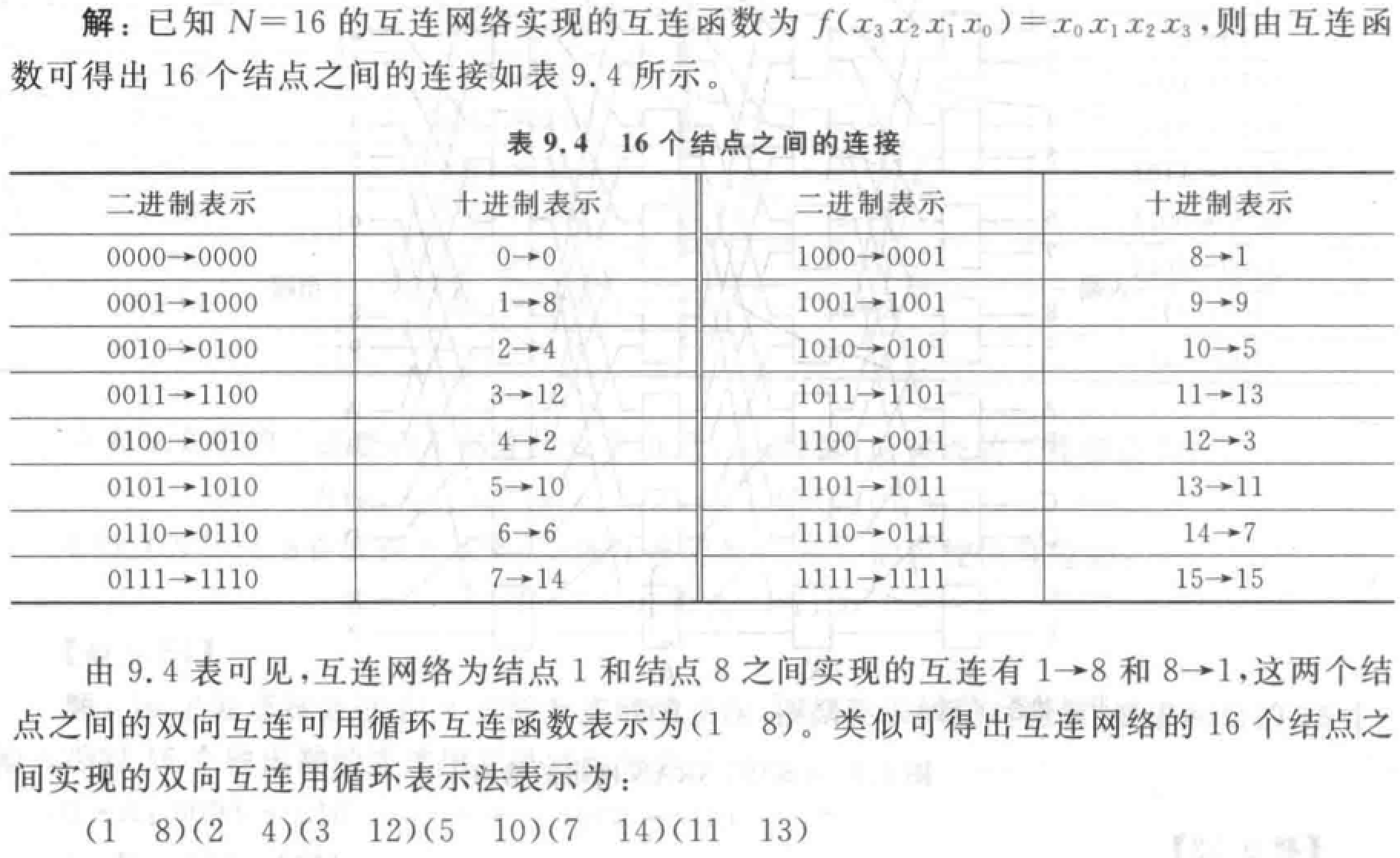• 恒等函数：$I(x_{n-1}x_{n-2}...x_1x_0) = (x_{n-1}x_{n-2}...x_1x_0)$

• 交换函数：用于构造立方体和超立方体互连网络。$Cube_1(x_{n-1}x_{n-2}...x_1x_0)=x_{n-1}x_{n-2}...\bar{x_1}x_0$ 实现二进制地址编号中第 $k$ 位互反的输入端和输出端之间的连接。互连函数种类为 $log_2N$$N$ 为结点个数。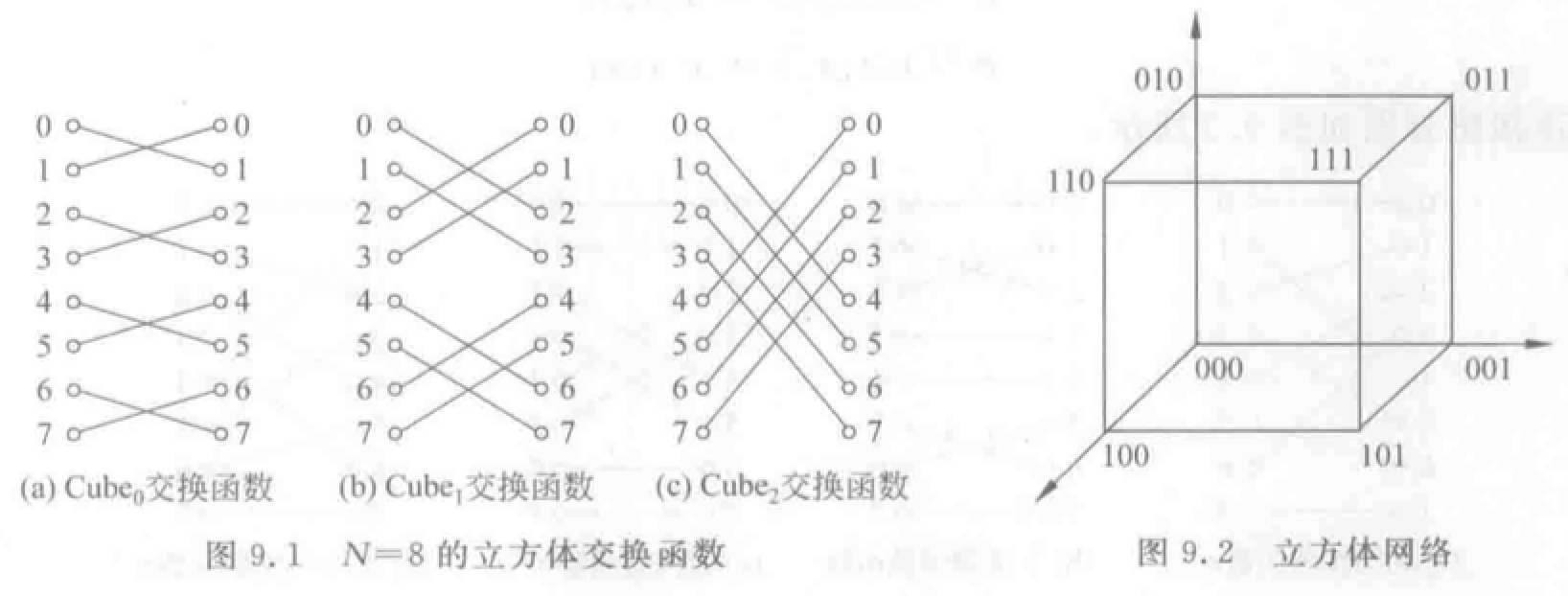• 均匀洗牌函数：用于构造 Omega 和逆 Omega 网络。将入线均分，前一部分和后一部分按顺序一个接一个交叉连接。

• 均匀洗牌：$\sigma(x_{n-1}x_{n-2}...x_1x_0)=x_{n-2}x_{n-3}...x_1x_0x_{n-1}$，将入线二进制地址循环左移一位得到。
• 逆均匀洗牌：$\sigma(x_{n-1}x_{n-2}...x_1x_0)=x_0x_{n-1}x_{n-2}...x_2x_1$，循环右移。
• $k$ 个子函数：把互连函数作用于低 $k$ 位。第 $k$ 个超函数：把互连函数作用于高 $k$ 位。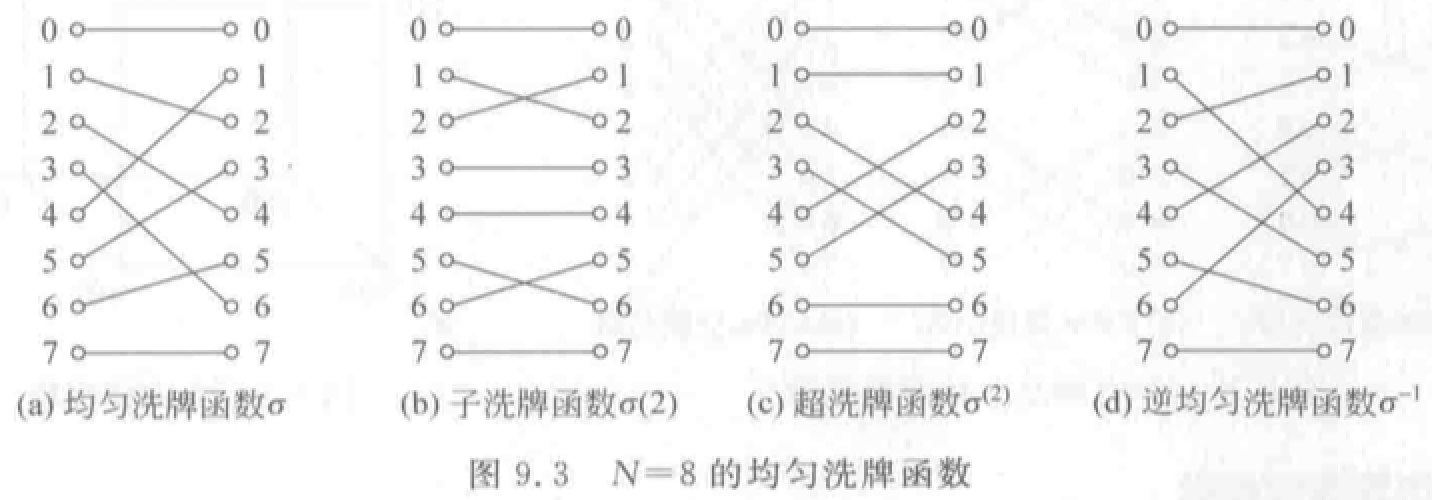• 蝶式函数：$\beta (x_{n-1}x_{n-2}...x_1x_0)=x_0x_{n-2}...x_1x_{n-1}$。将输入端二进制最高位 $x_{n-1}$ 与最低位 $x_0$ 互换位置得到。

• 反位序函数：$\rho (x_{n-1}x_{n-2}...x_1x_0)=x_0x_1...x_{n-2}x_{n-1}$，把输入二进制编号各位次序颠倒。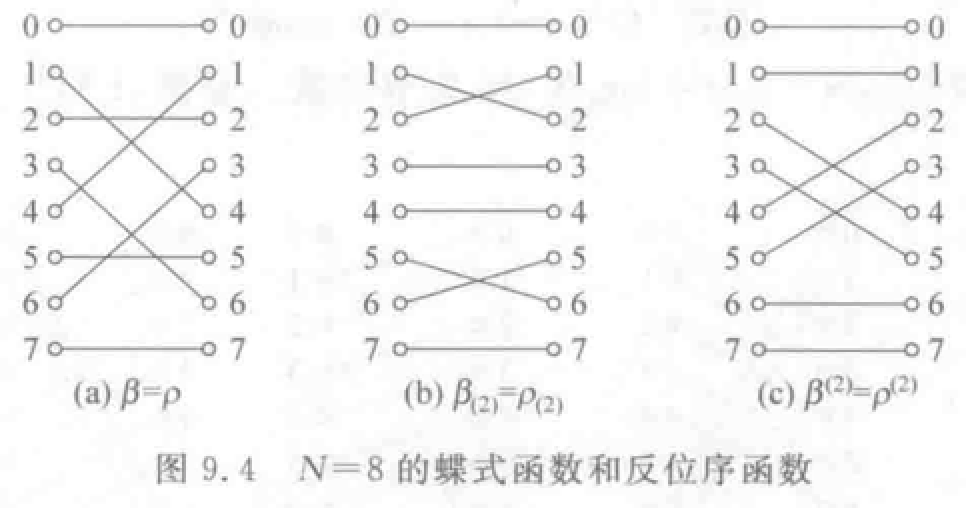• 移数函数：$\alpha (x) = (x \pm k)\mod N$ 把二进制编号模 $N$• $PM2I$函数：加减 $2^i$。构成数据变换网络的基础。实质为 1、2、4 个环形网。

• $PM2_{+i}(x) = (x + 2^i)\mod N$$0 \le i \le log_2N$
• $PM2_{-i}(x) = (x - 2^i)\mod N$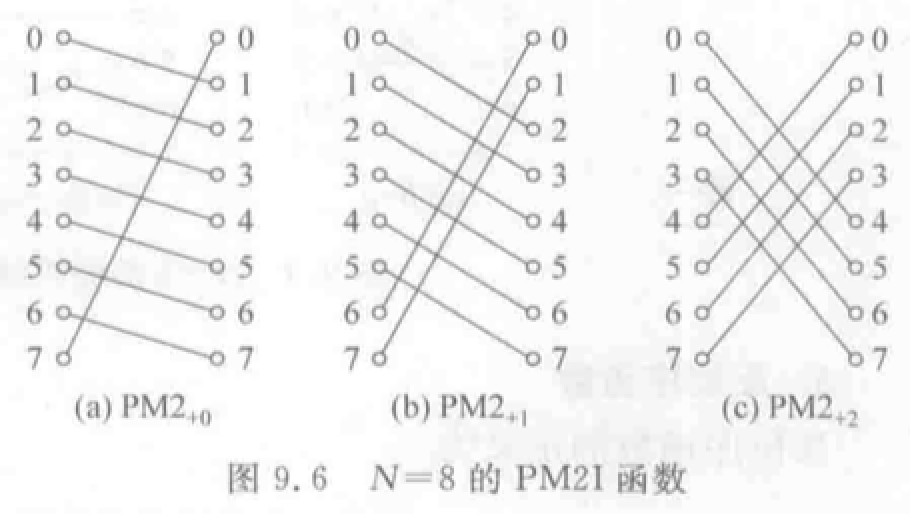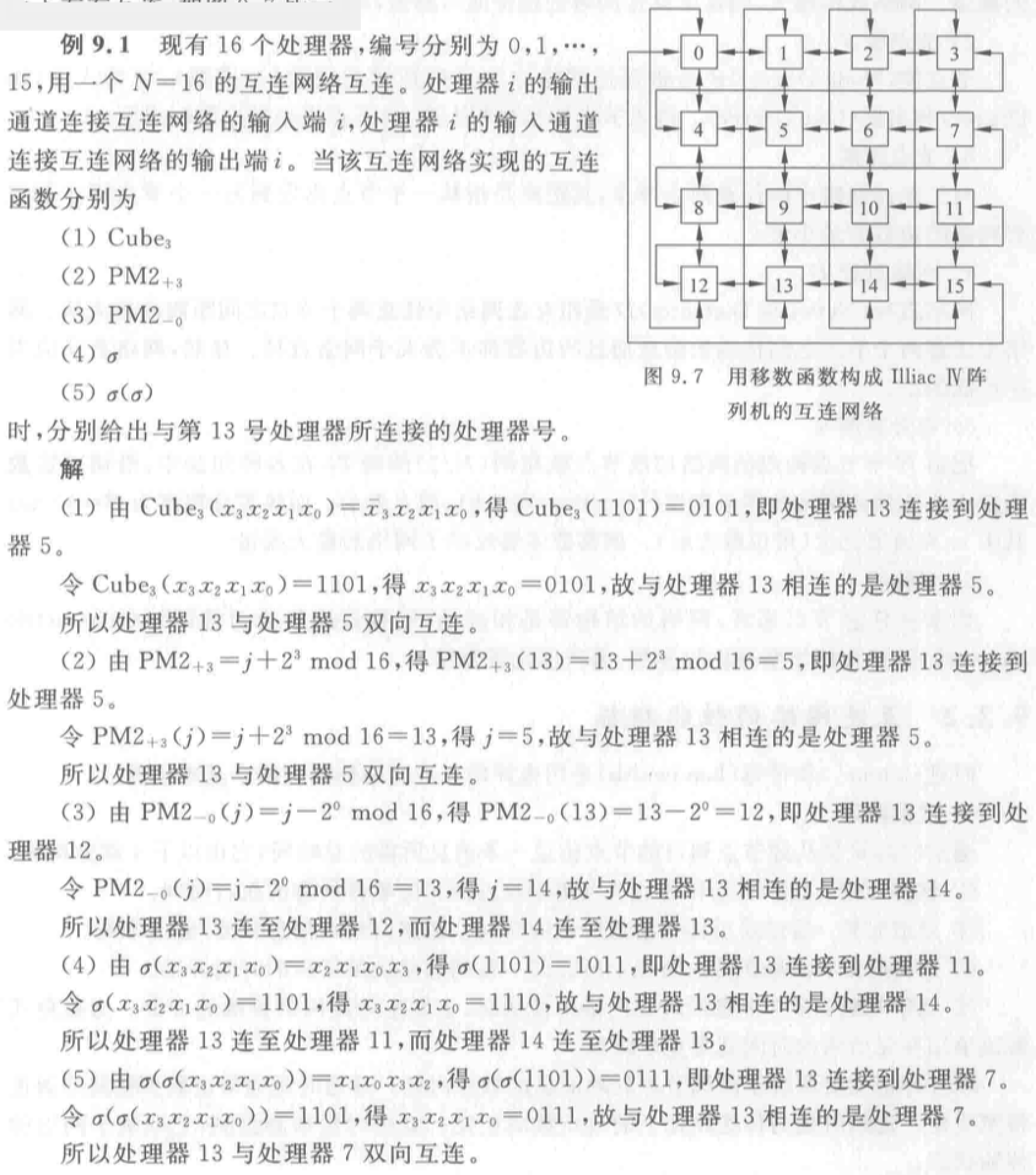## 结构参数与性能指标

6 个结构参数：

• 网络规模 $N$
• 结点度 $d$：结点所连接的边数。（入度、出度）
• 结点距离：从一个结点出发到另一个结点，经过边数的最小值。
• 网络直径 $D$：网络中结点距离的最大值。（越小越好）
• 等分宽度 $b$：把网络均分为结点数相同的两半，在各种切法中，沿切口边数的最小值。线等分宽度 $B = b \times w$$w$ 为通道宽度（bit），等分宽度反映网络最大流量。
• 对称性：从任意结点看，网络的结构都是相同的。

• 时延：
• 通信时延
• 软件开销：源节点、目的结点收发消息软件的执行时间。取决于软件内核。
• 通道时延：消息长度/通道带宽。通常由瓶颈段带宽决定。
• 选路时延：与传送路径上结点数成正比。
• 竞争时延：避免、解决争用所需的时间。很难预测，取决于网络状态。
• 网络时延：通道时延+选路时延。由网络硬件决定，与软件、传输状态无关。
• 带宽
• 端口带宽：单位时间内从该端口传输到其他端口的最大信息量。
• 对称网络中各端口带宽相等=网络端口带宽。
• 非对称网络的端口带宽=所有端口带宽的最小值。
• 聚集带宽：网络从一半节点到另一半节点，单位时间传送的最大信息量。
• 等分带宽：与等分宽度对应的切平面中，所有边合起来，单位时间传送的最大信息量。

## 静态与动态互连网络

### 静态互连网络

• 线性阵列：一维线性网络，$N$ 个结点用 $N-1$ 个链路连成一行。

• 环和带弦环：用一条附加链路将线性阵列端结点连接，构成环。

• 带弦环：增加链路越多，结点度越高，网络直径越小。
• 全连接网络：极端情况。
• 循环移数网络：在环上每个结点到所有与其距离为 2 的整数幂的结点之间都增加一条附加链路。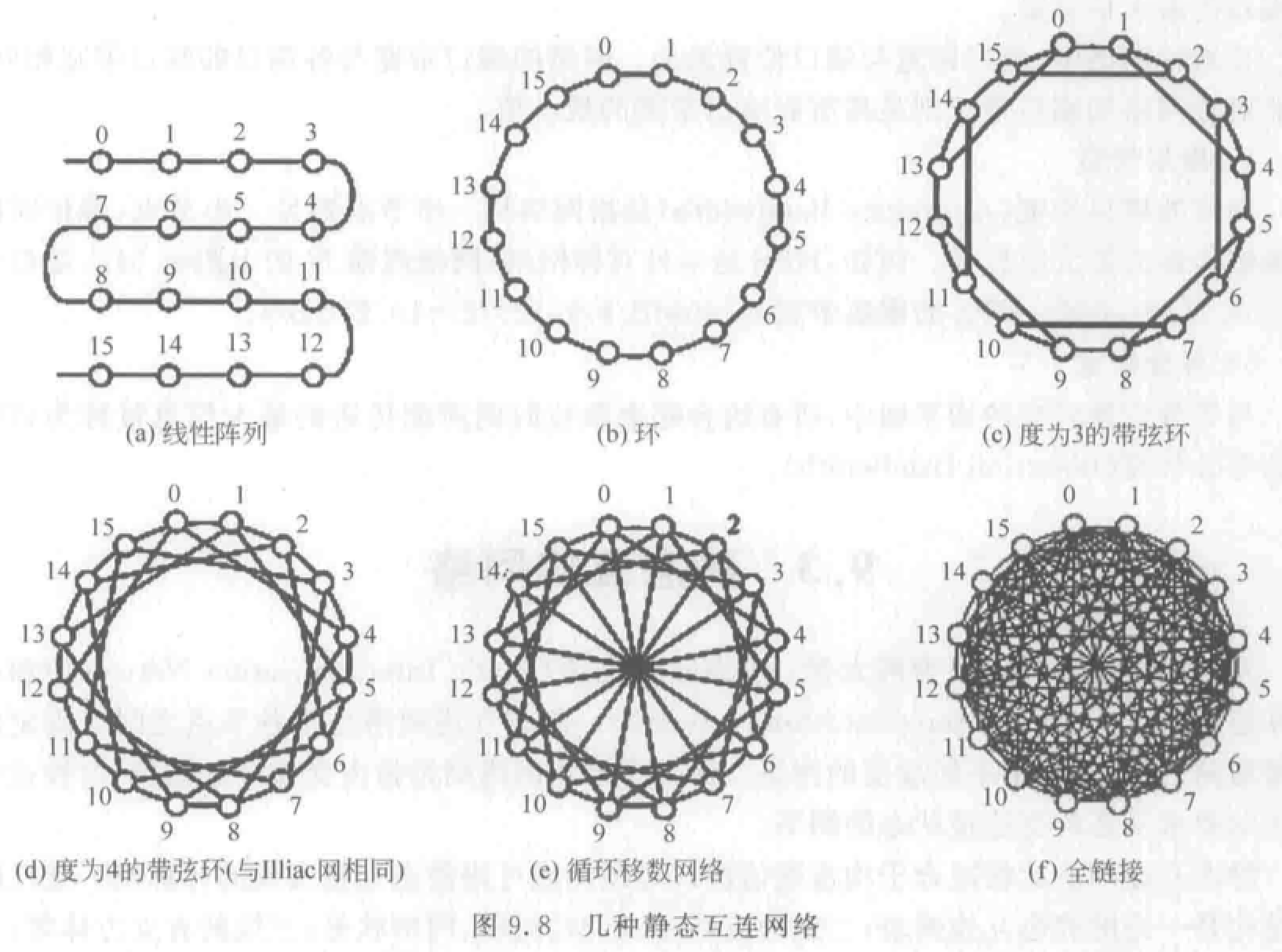• 树状：$k$ 层完全平衡二叉树有 $N=2^k-1$个结点。

• 星形：CS 模式。中心结点故障，整个系统瘫痪。

• 胖树形：越靠近树根，树干越粗（通道带宽增加）。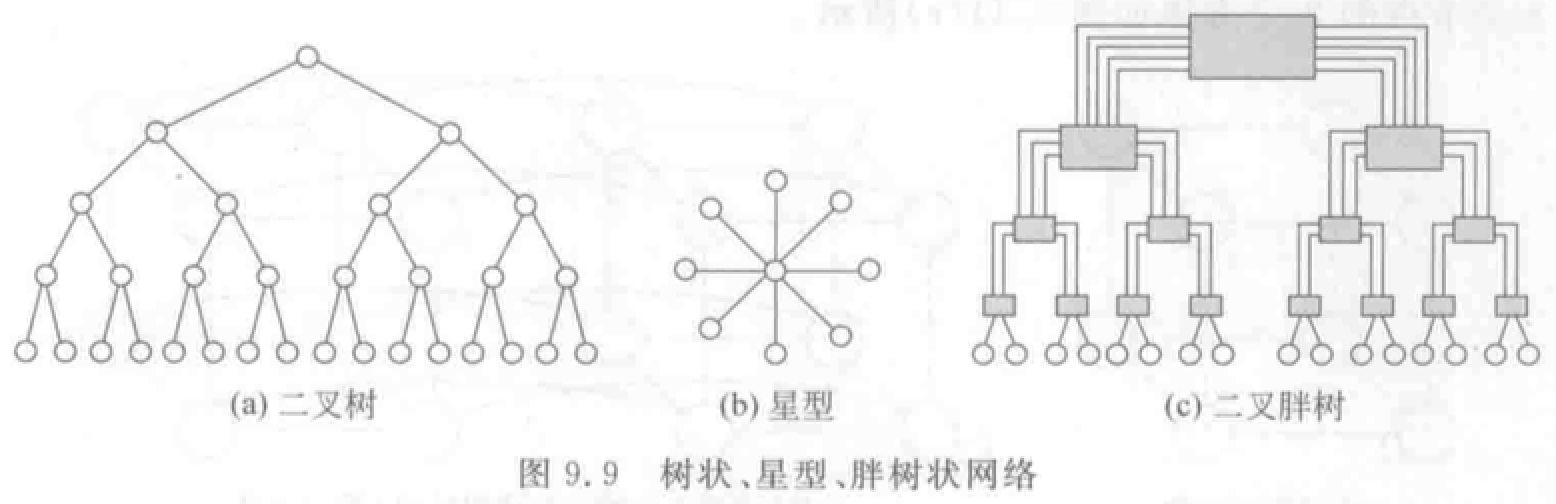• 网格形、Illiac、环状网络：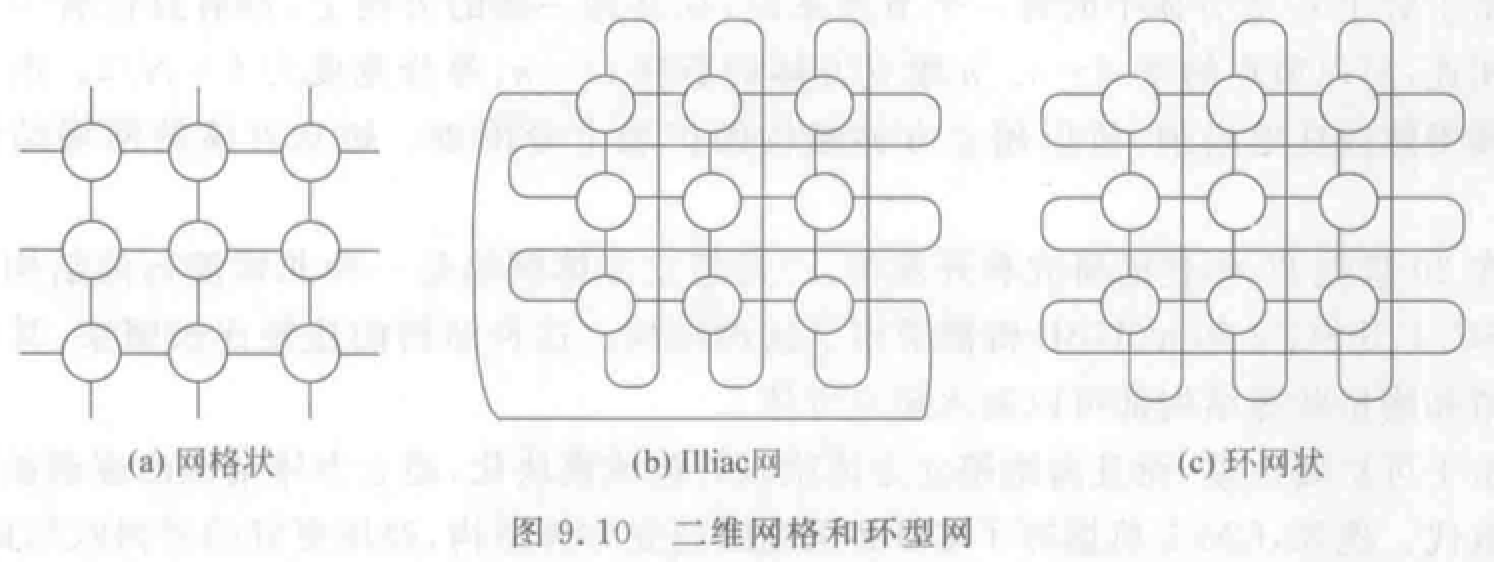• 超立方体：$n$ 维结点数 $N=2^n$。对于一个结点，在每一维方向上都只和一个结点相连。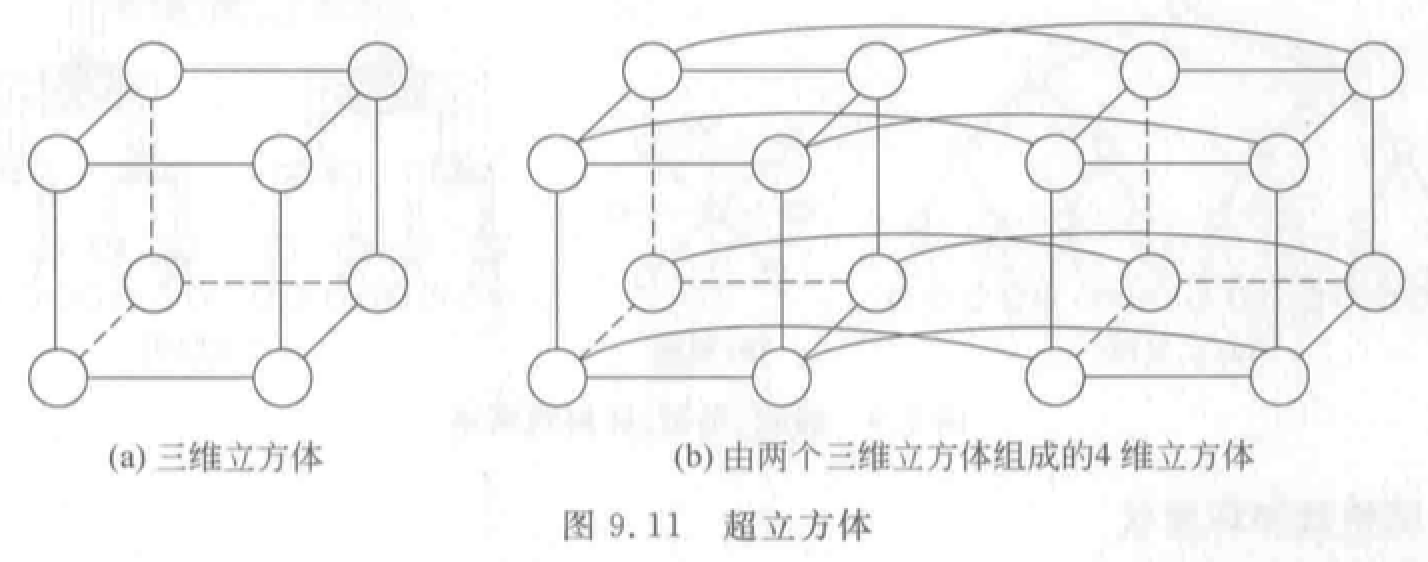• 带环 $n$-立方体（$n$-CCC）：用 $k$ 个结点构成的环代替 $k$ 维超立方体。结点度恒定，扩展性好。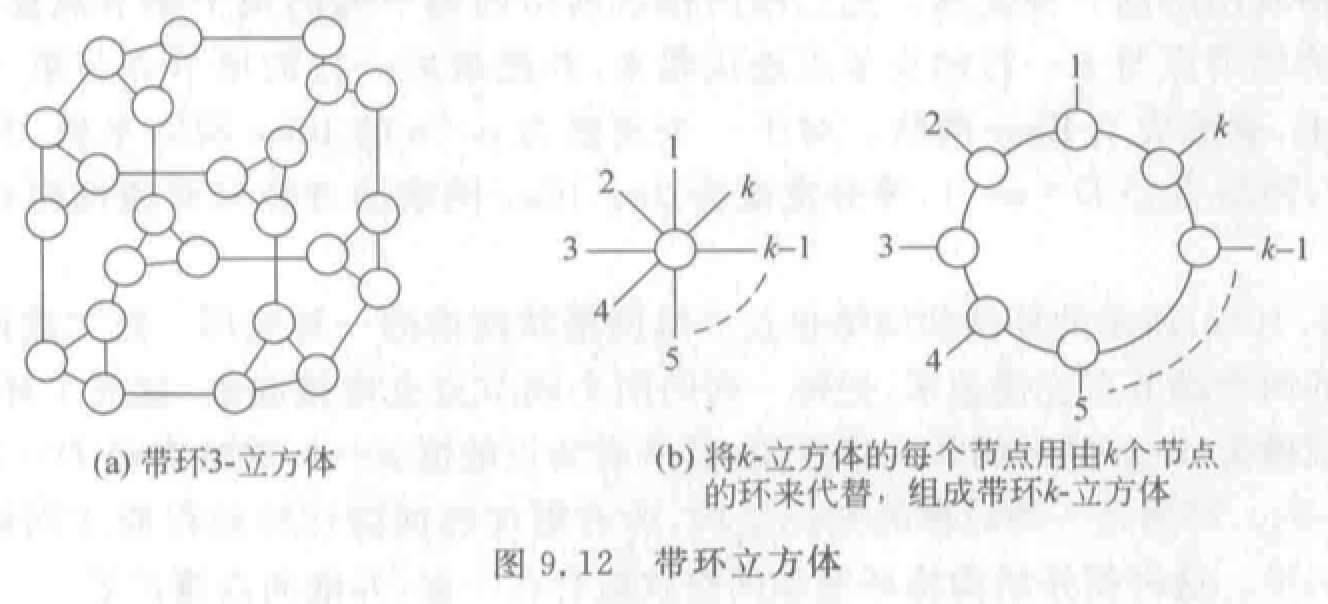• $k$$n$-立方体网络：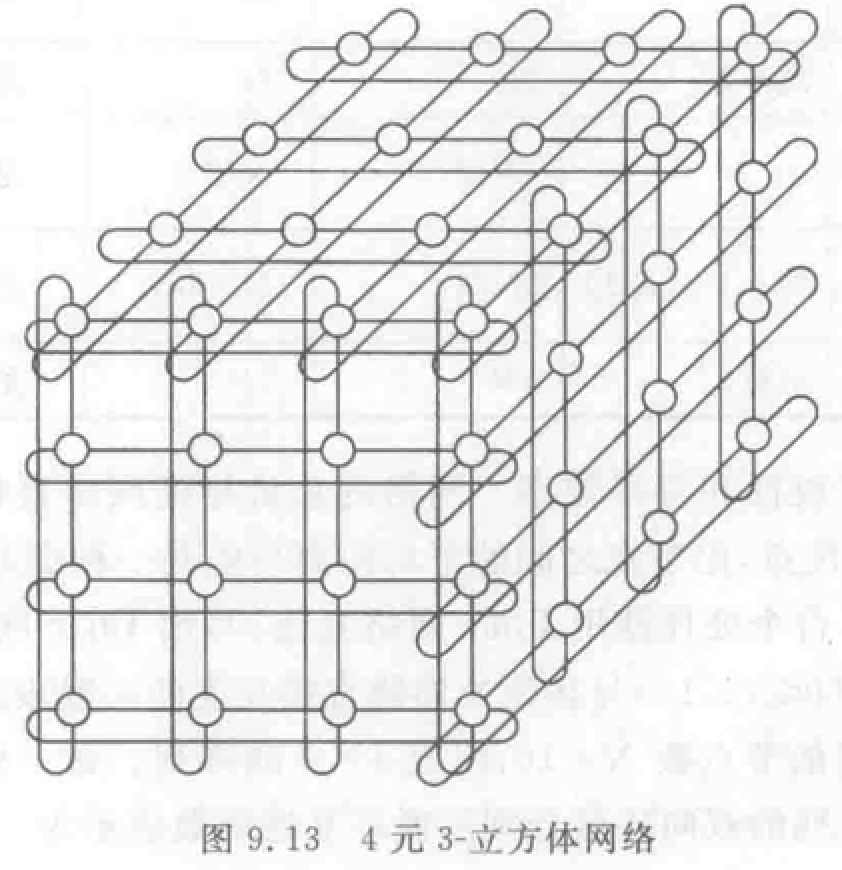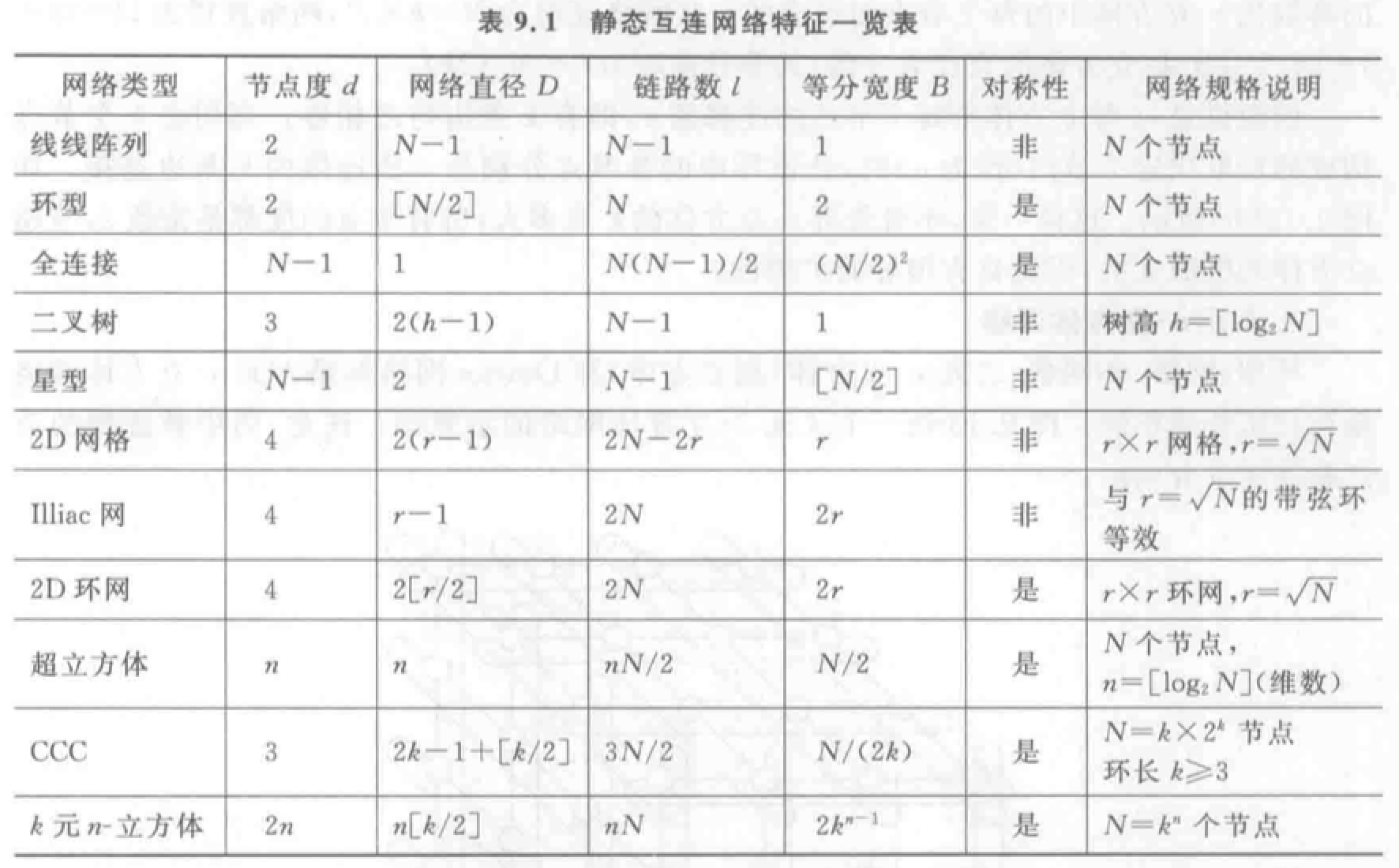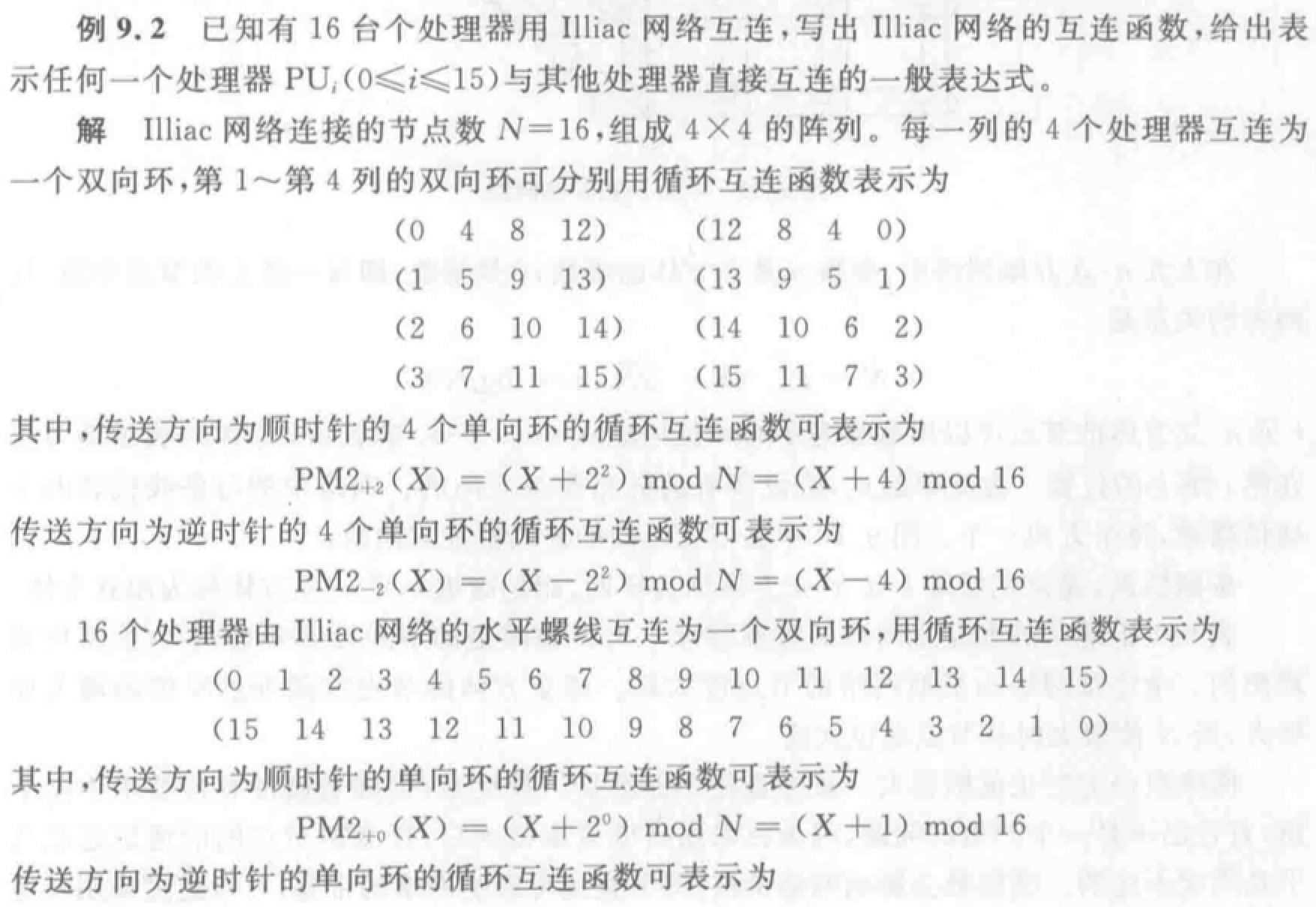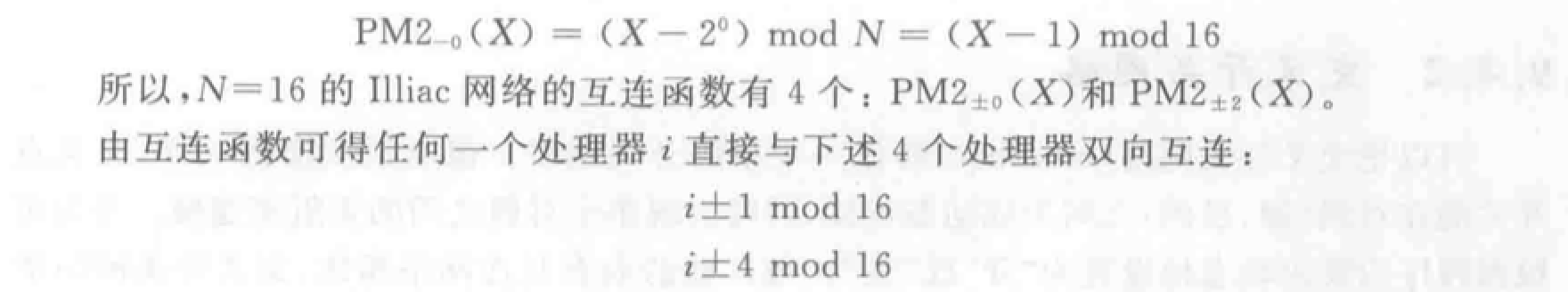### 动态互连网络

• 总线结构

• 优点：结构简单、实现成本低。
• 缺点：模块分时共享，带宽较窄。（可采用多总线或多层次总线解决）
• 交叉开关网络

• 可以无阻塞实现 $n!$ 种置换。
• 其他参考交换原理。
• 多级互联网络

• MIMD 和 SIMD 计算机都采用多级互连网络 MIN。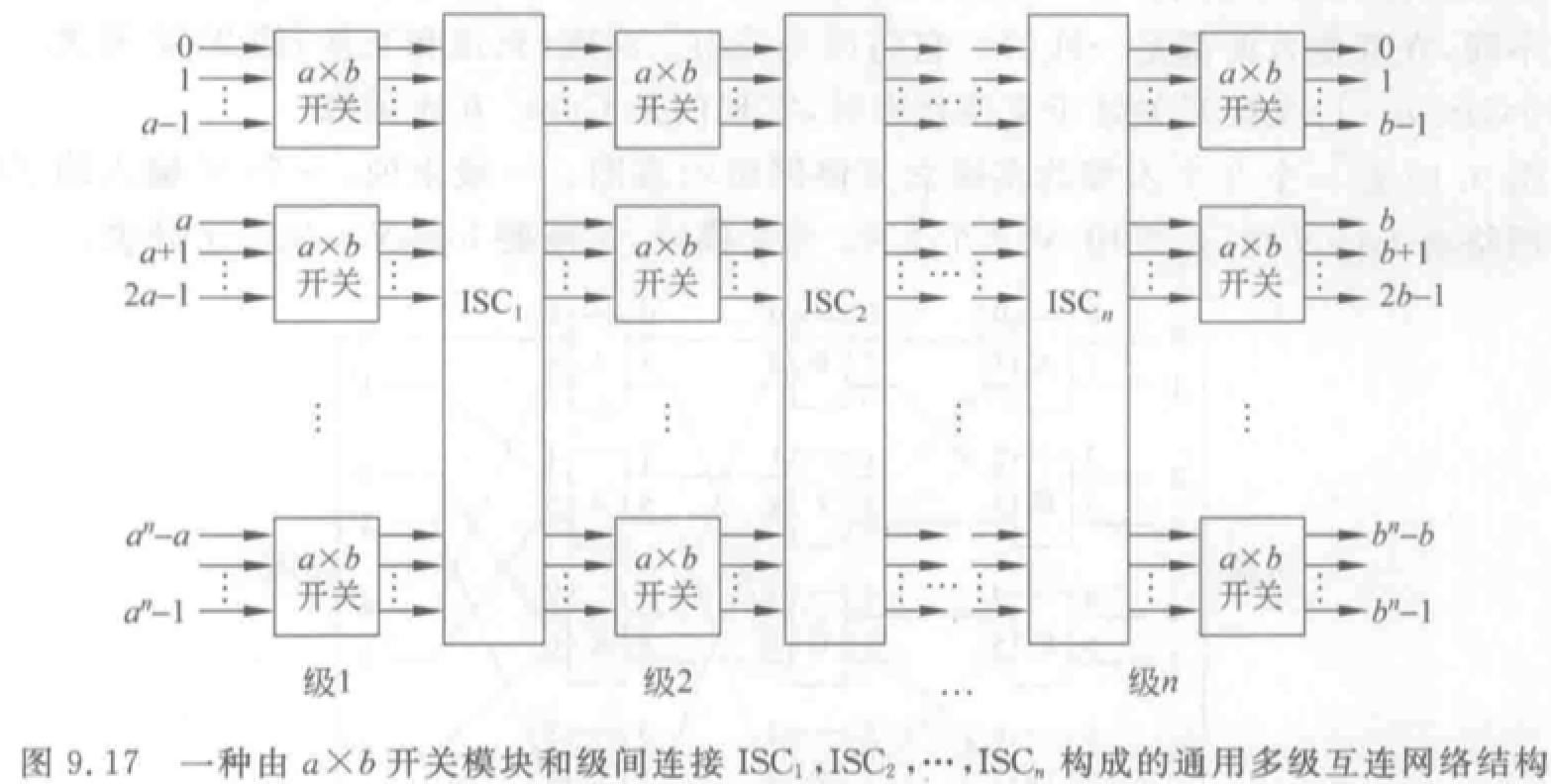• 区别：开关模块、控制方式、级间互连模式不同。

• $2\times2$ 开关模块：直送、交叉、上播、下播。
• 控制方式：级控制、单元控制、部分级控制
• 级间互连：参考交换原理。
• 多级立方体网络：STARAN 网络、间接二进制 $n$ 方体网络。都采用二功能（直送和交换）的 $2\times2$ 开关。当第 $i$ 级（$0\leq i\leq n-1$）交换开关处于交换状态时，实现的是 $Cube_i$ 互联函数

STARAN 网络采用级控制（实现交换功能）和部分级控制（实现移数功能），间接二进制 $n$ 立方体网络采用单元控制。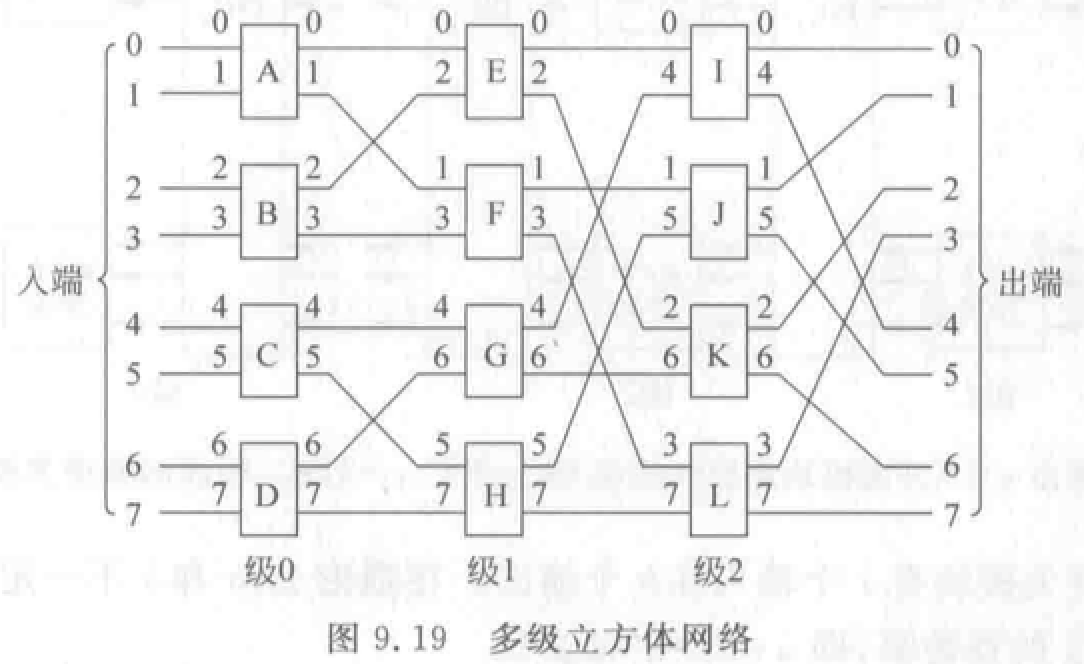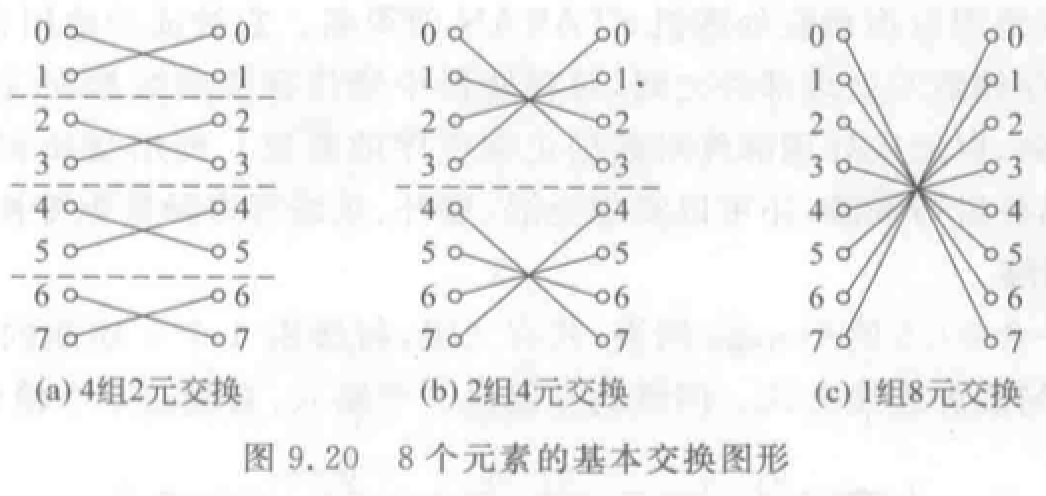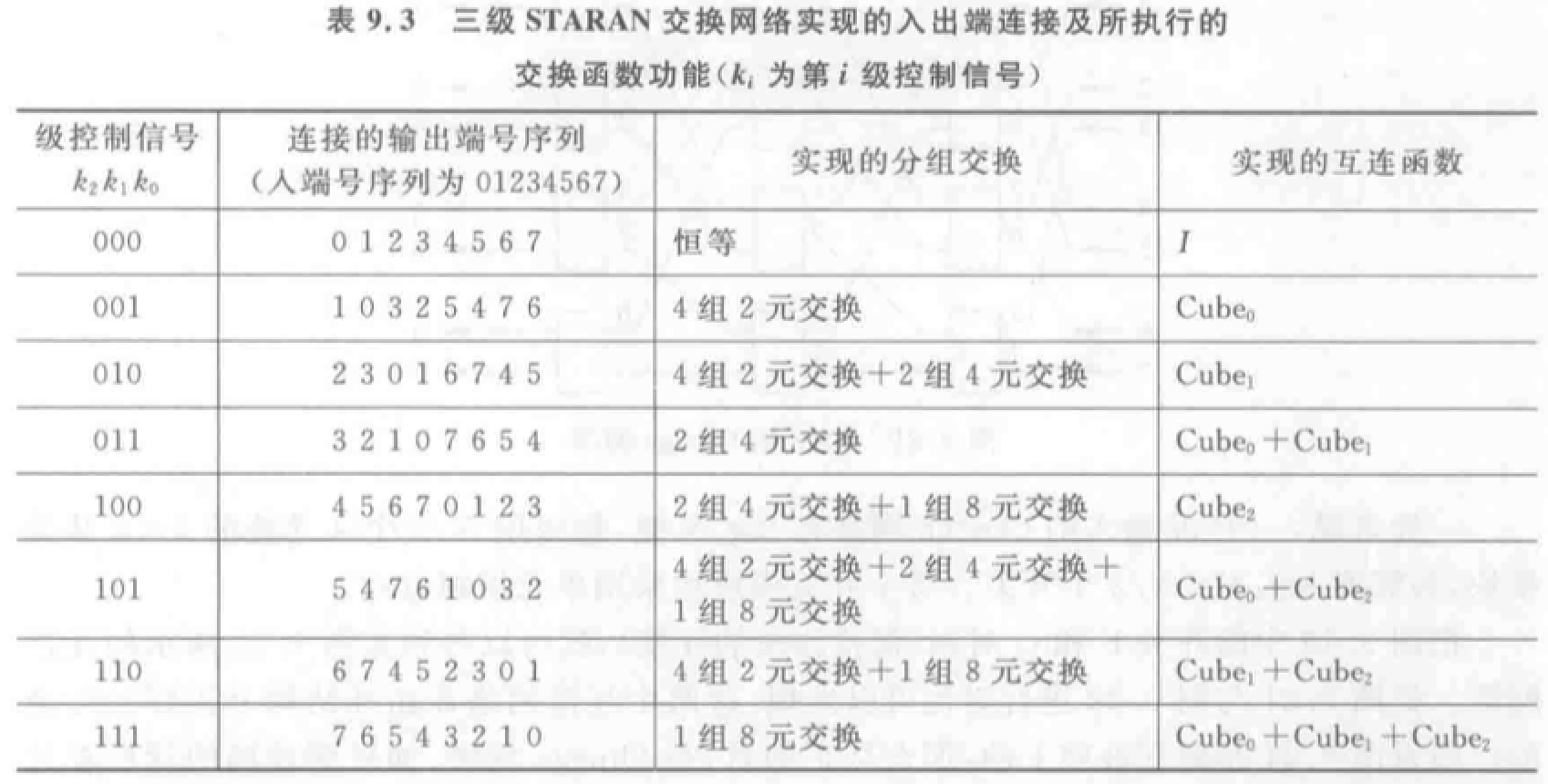例题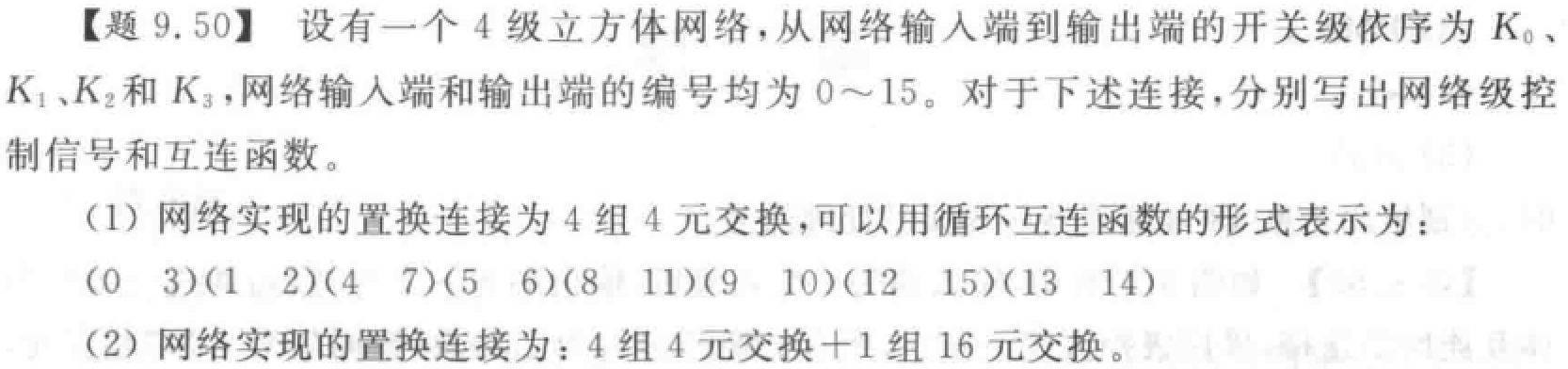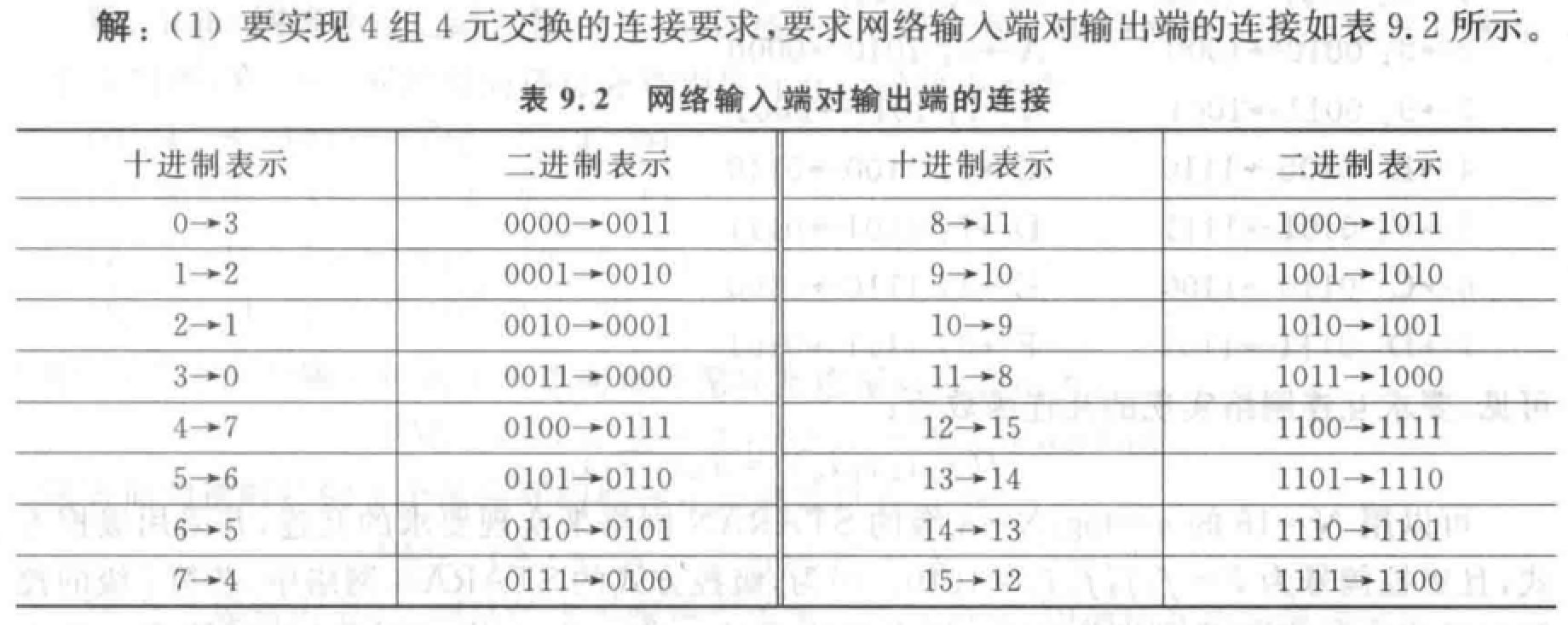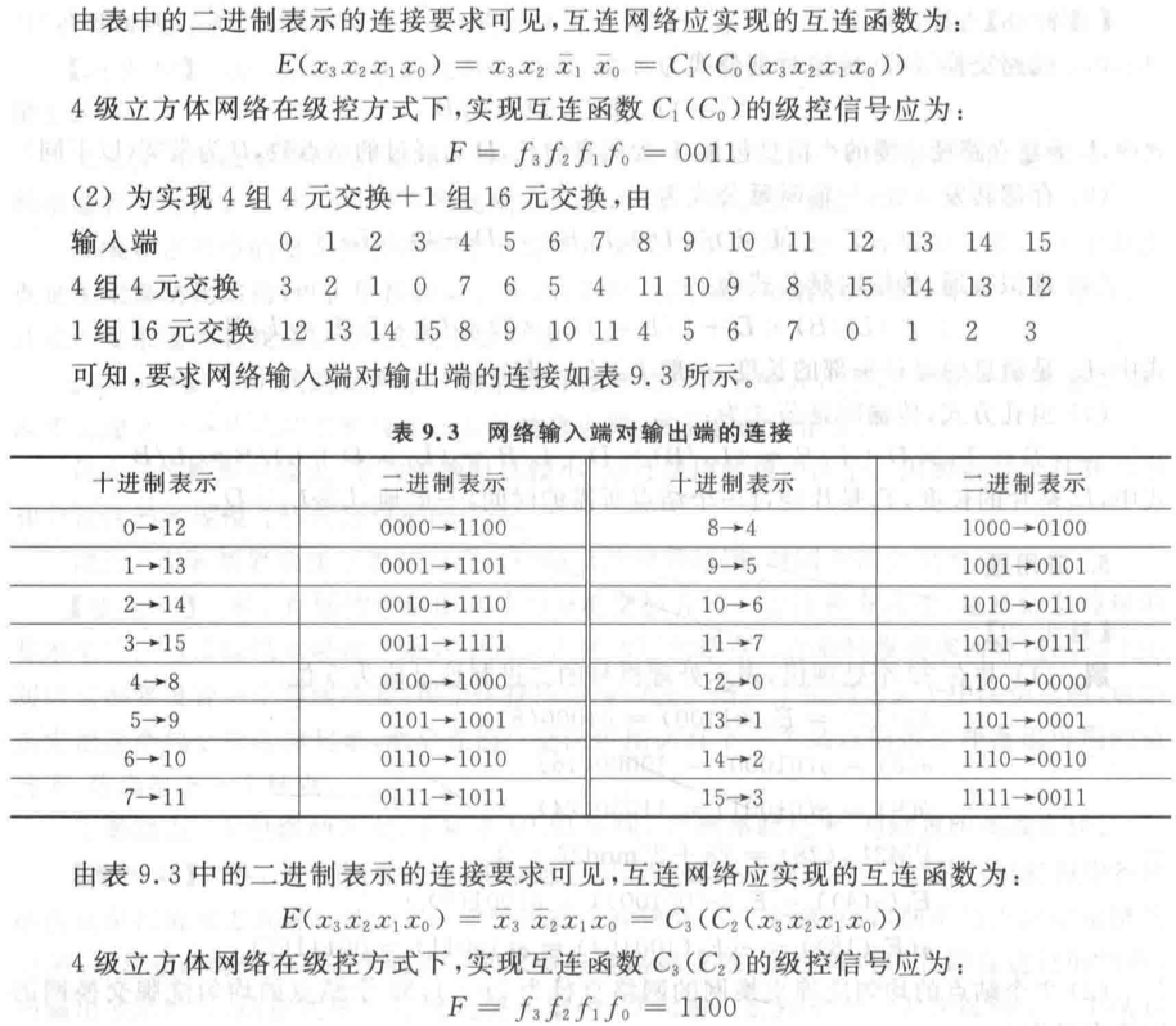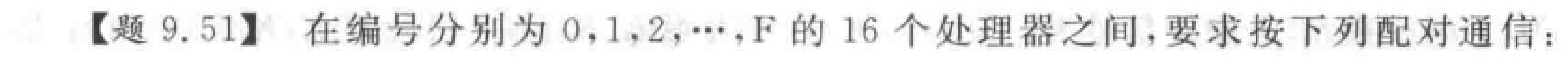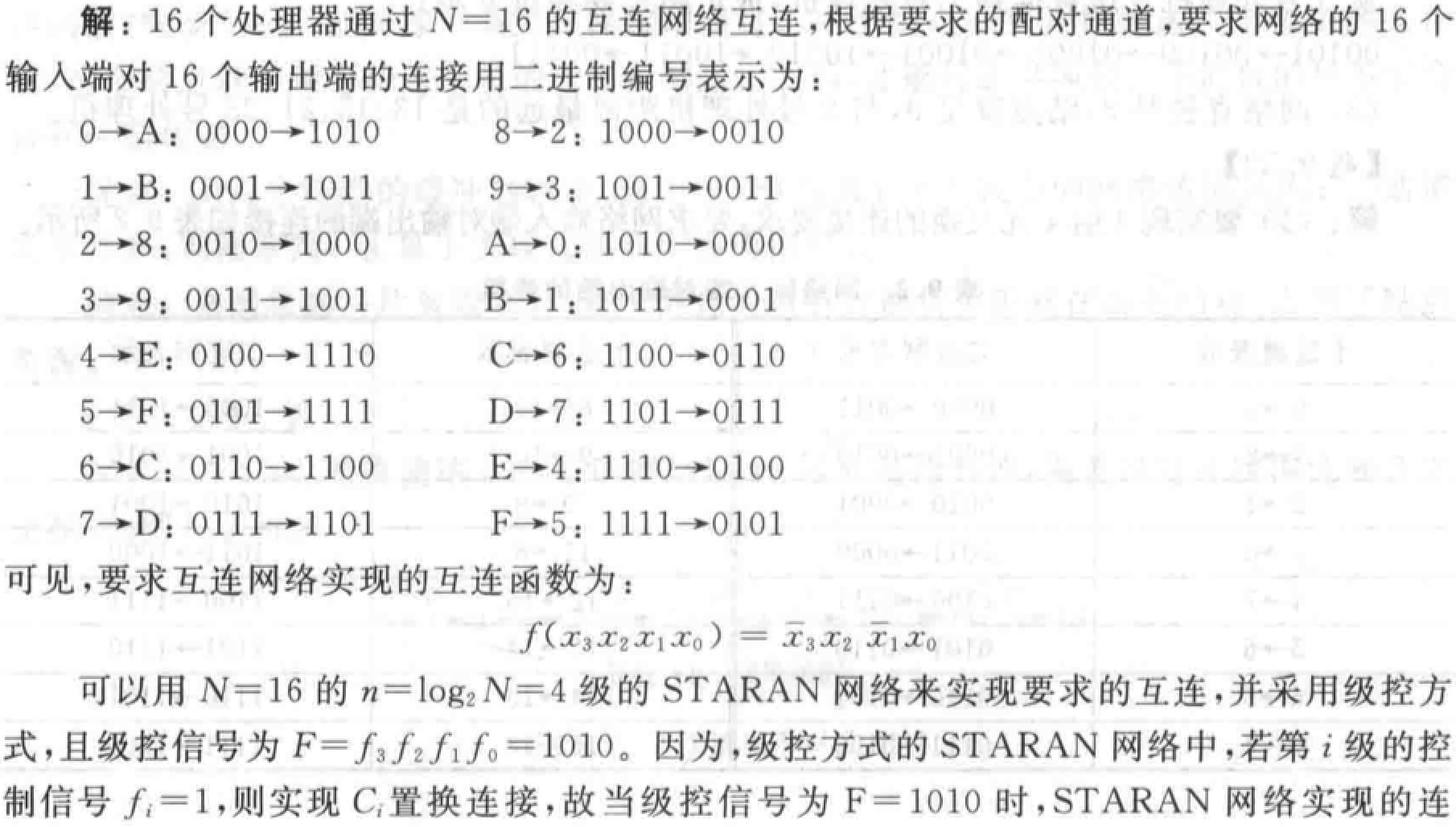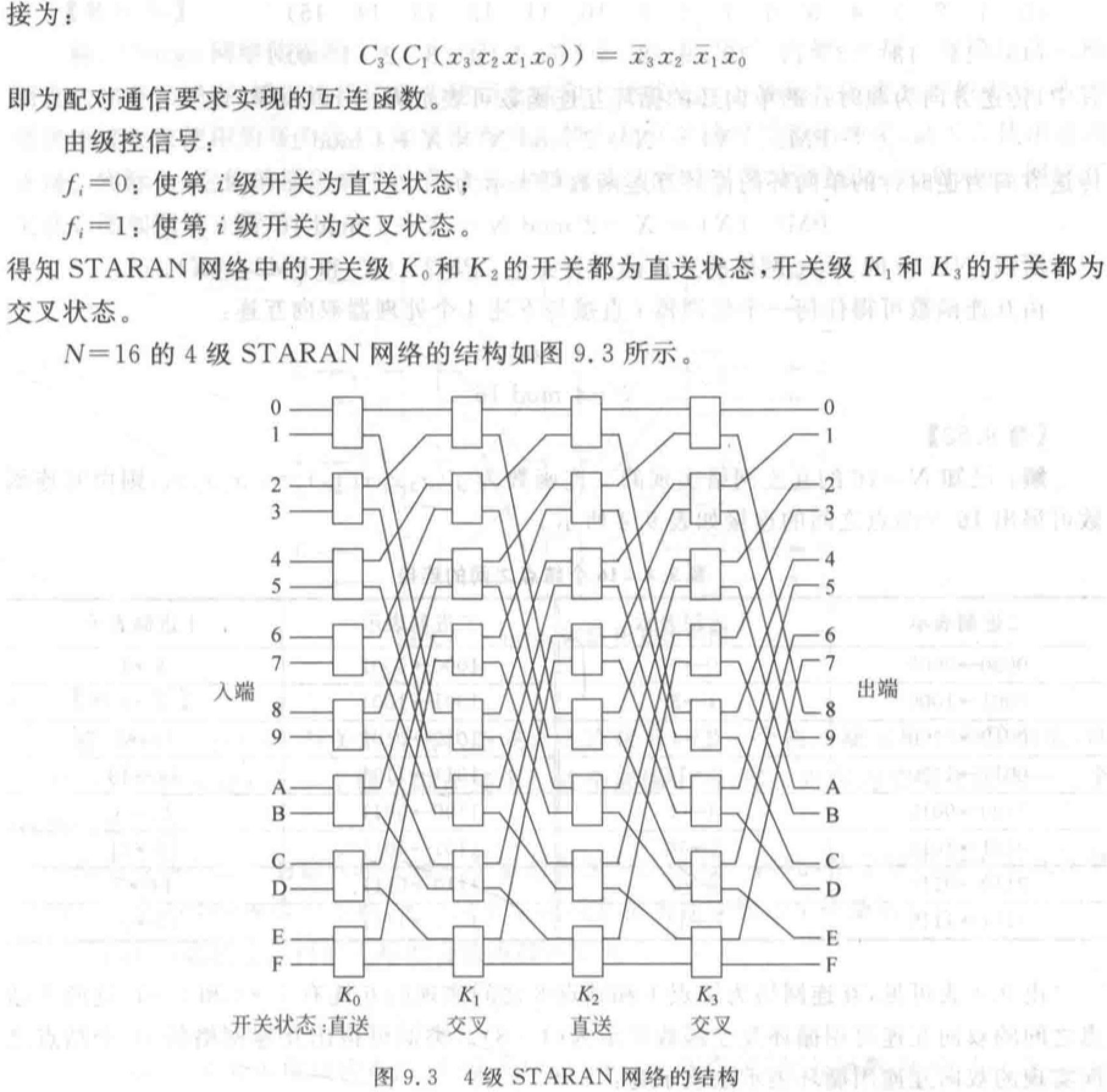• Omega 网络：级间全部采用均匀洗牌。$N=8$ 的多级立方体互连网络的另一种画法。

$N$ 个输入有 $\log_2 N$级，每级 $N/2$ 个四功能的 $2\times2$ 开关模块。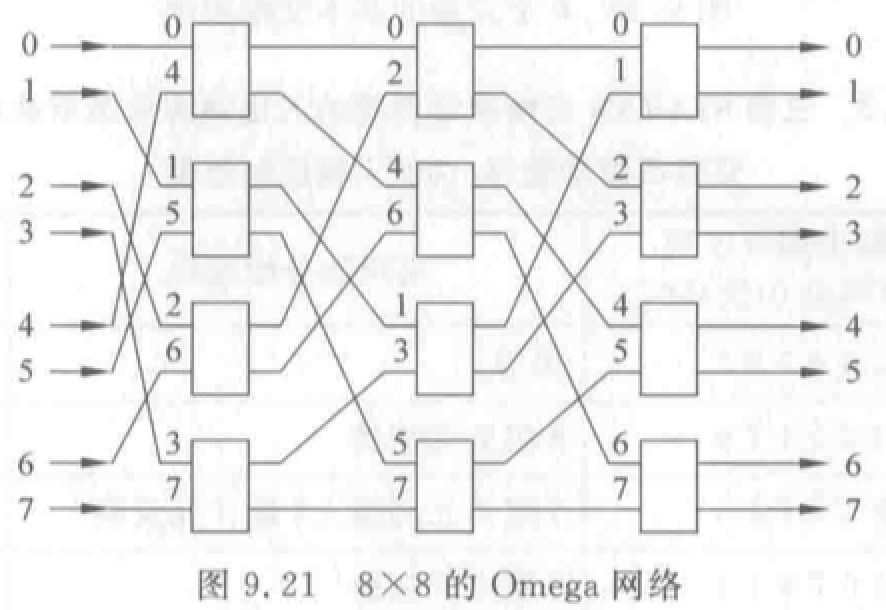例题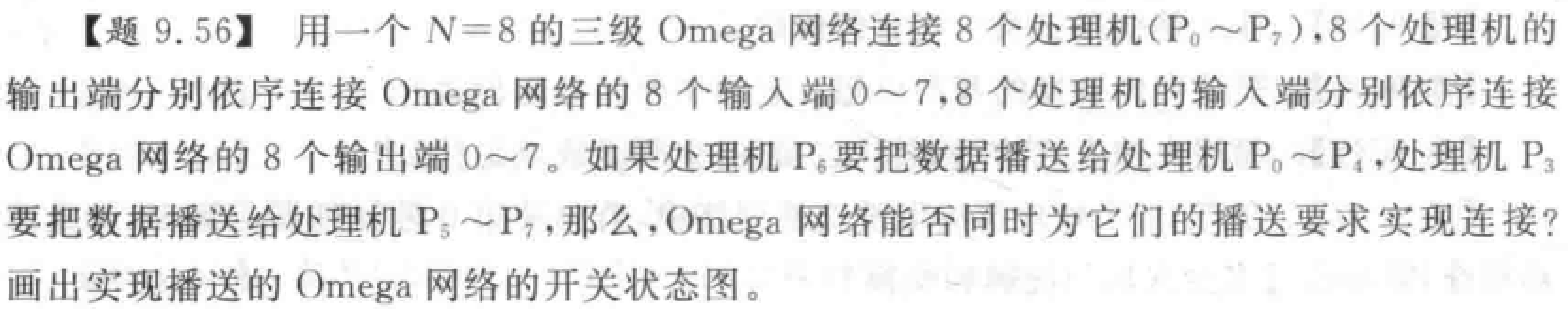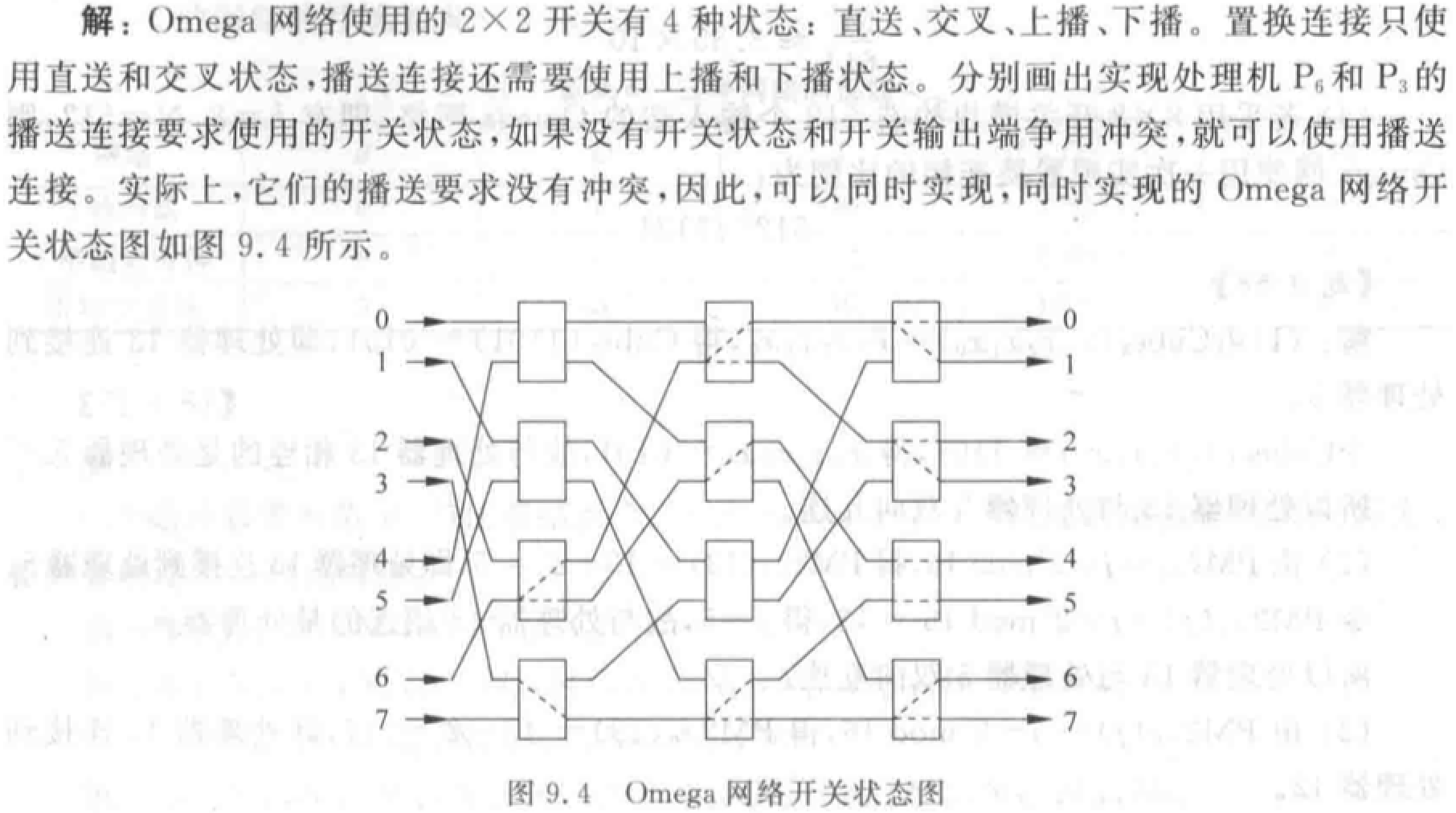（1）N 个输入的不同排列数为 $N!$

（2）N 个输入端、输出端的 Omega 网络有 $n＝log_2N$ 级开关级，每级开关级有 $N/2$$2\times 2$ 的 4 功能开关，总共有 $(N/2)\log_2N$ 个开关。置换连接是指网络的输入端与输出端的一对一连接，故只考虑 $2\times 2$ 开关的 2 个功能状态，即直送与交叉。网络采用单元控制，因此，每个开关都根据连接要求处于 2 个功能状态中的一种状态，所以，由 $(N/2)\log_2N$ 个开关组成的 Omega 网络的开关状态的种数为 $2^{(N/2)\log_2N}=N^{N/2}$

（3）若 $N=8$，则一次通过能实现的置换数占全部排列的百分比为 $\frac{N^{N/2}}{N!}=10.16\%$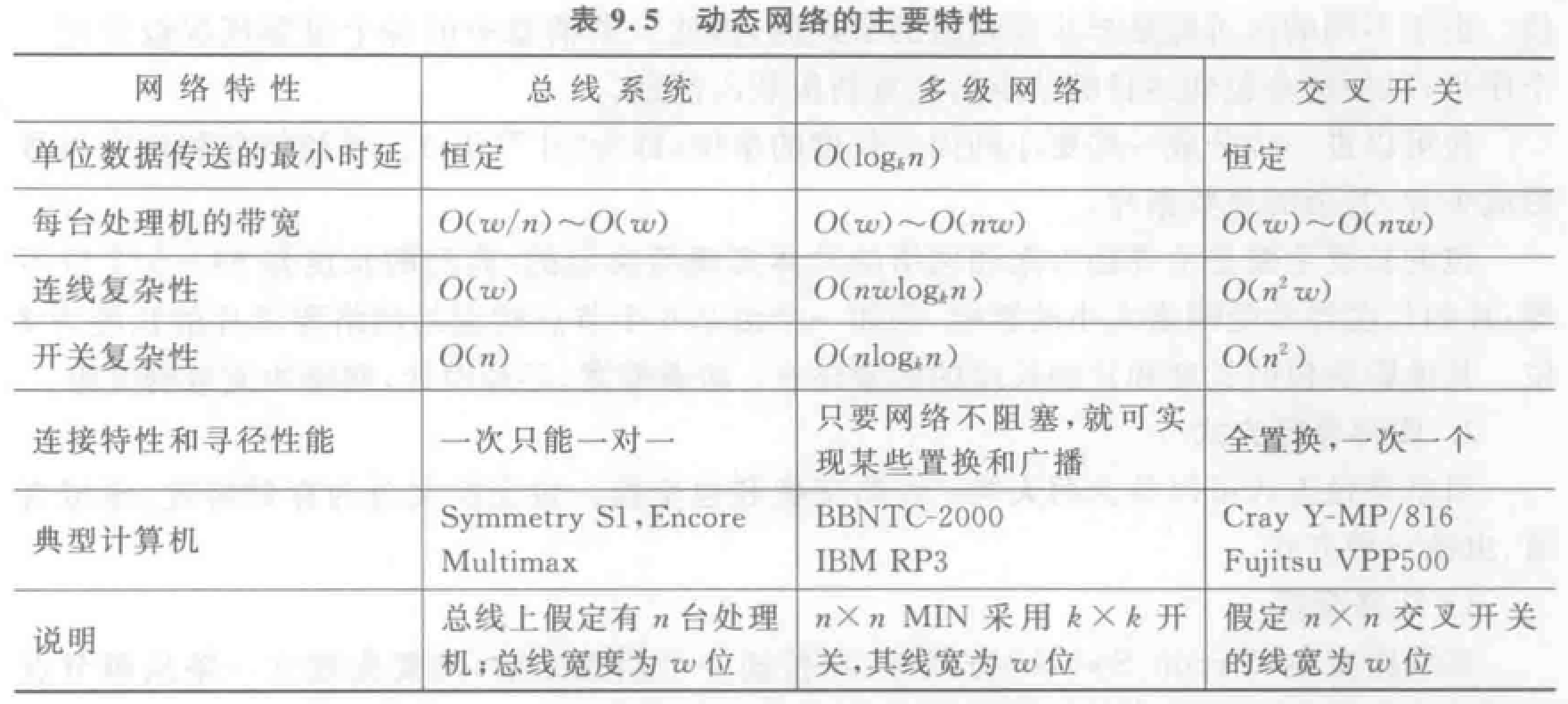## 消息传递机制

RPC：Remote Process Call，远程进程调用。

• 消息格式：
• 消息：节点间通信的逻辑单位，由若干“包”组成。包的长度固定但是数量可变。包是包含寻径所需目的地址的基本单位。包分为若干“片”，大小固定。寻径信息和包序列号形成头片，其余是数据片。
• 两类四种寻径方式
• 线路交换：建立物理通路再传递信息。时延 $T=\frac{L+L_t \times (D+1)}{B}$$L$ 为包总长，$L_t$ 为建立路径所需最小包长度，$D$ 为中间结点个数，$B$ 为带宽。
• 优点：带宽大，平均时延小，占用缓冲区小。
• 适合动态和突发性的大规模并行处理数据传送。
• 缺点：频繁建立物理通路时间开销大。
• 包交换
• 存储转发：$T_{SF} = \frac{L}{B}(D+1)$
• 优点：简单。
• 缺点：包缓冲区大、网络时延大。
• 虚拟直通路：$T = T_f \times D + \frac{L}{B} \approx \frac{L}{B}$
• 优点：通信时延与结点数无关。
• 缺点：中间结点依然需要缓冲器、寻径阻塞时依然需要存储整个包，占用缓冲区大。
• 虫蚀：最小单位为片。一个结点把头片送到下一个结点后，后面的各片才能依次送出。$T_{WH} \approx \frac{L}{B}$
• 优点：每个结点缓冲器小，易于 VLSI 实现。有较小的网络传输延迟。
• 缺点：一片被阻塞时，该消息所有片都被阻塞在结点。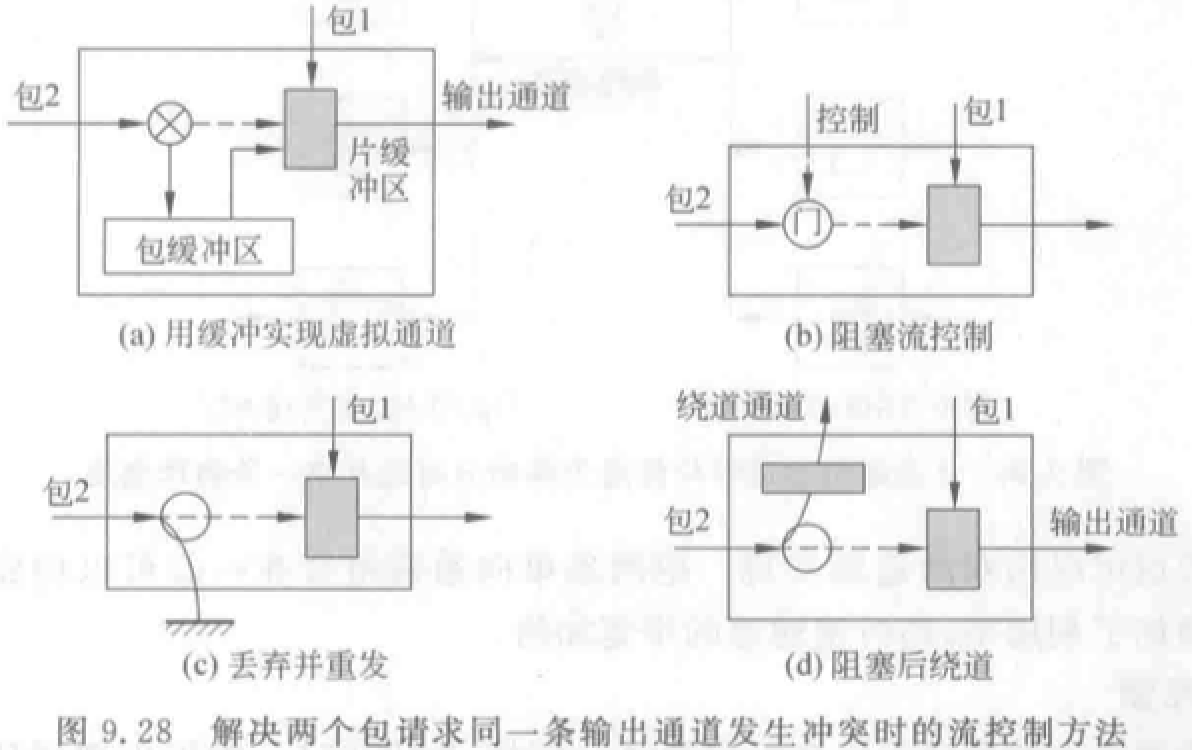• 单播：对应于一对一的通信情况，即一个源结点发送消息到一个目的结点。
• 选播：对应于一到多的通信情况，即一个源结点发送同一消息到多个目的结点。
• 广播：对应于一到全体的通信情况，即一个源结点发送同一消息到全部结点。
• 会议：对应于多到多的通信情况。
• 通道流量可用传输有关消息所使用的通道数来表示。
• 通信时延则用包的最大传输时间来表示。

# 第十章 多处理机

• MIMD 计算机的特点、分类：PVP/SMP/MPP/DSM/COW

• Cache 一致性问题成因和解决方法（写作废、写更新）
• 监听协议法
• 目录表协议（三种结构）

## MIMD 计算机特点、分类

MIMD 是通用计算机系统的主要选择。

• 并行向量多处理机 PVP，第四章：多台巨型向量处理机，共享存储器互连而成。
• 大规模并行处理机 MPP，第十章：计算机模块作为一个结点，通过专用接口连接到互连网络上。
• 集中式共享存储器多处理机 SMP：主流。
• 分布式存储器多处理机。
• 分布式共享存储器系统 DSM。
• 机群多处理机 COW，第十二章：多个完整的计算机，通过商品化互连网络（以太网、ATM 等）相连。

• 集中式共享存储器结构（对称式共享存储器多处理机 SMP）：

• 共享集中式物理存储器、通过总线或交换开关互连。
• 也被称为 UMA（Uniform Memory Access）结构。各处理器访问存储器时延相同。
• 最流行结构。
• 分布式存储器多处理机：存储器分布在各结点。

• 要求高带宽互连网络。
• 优点：① 若大多数需求为本地访存，可以降低存储器、互连网络带宽需求。② 本地存储器访问时延小。
• 缺点：① 处理器之间通信复杂。② 处理器之间访问延迟较大。

分布式存储器多处理机两种存储器系统结构：

• 共享地址空间（每个结点存储器统一编址）：分布式共享存储器系统 DSM， 或非均匀访存模型 NUMA
• 共享逻辑地址空间。
• 任意存储器可访问共享空间中任一单元。
• 不同处理器上同一物理地址指向同一存储单元。
• 具有共享存储器通信机制。采用访存类指令对地址进行读写。优点：
• 与常用的对称式多处理机使用的通信机制兼容。
• 易于编程，同时在简化编译器设计方面也占有优势。
• 采用大家所熟悉的共享存储器模型开发应用程序，而把重点放到解决对性能影响较大的数据访问上。
• 当通信数据量较小时，通信开销较低，带宽利用较好。
• 可以通过采用 Cache 技术来减少远程通信的频度，减少了通信延迟以及对共享数据的访问冲突。
• 在共享存储器上支持消息传递相对简单。
• 独立地址空间（编址相互独立）：多存在于机群 COW
• 系统地址空间由多个独立地址空间组成。
• 每个结点存储器只能本地存储器访问。
• 每个存储器-处理器模块是一台单独计算机
• 具有消息传递通信机制。采用显式传递消息请求操作或数据完成通信。优点：
• 硬件较简单。
• 通信是显式的，因此更容易搞清楚何时发生通信以及通信开销是多少。
• 显式通信可以让编程者重点注意并行计算的主要通信开销，使之有可能开发出结构更好、性能更高的并行程序。
• 同步很自然地与发送消息相关联，能减少不当的同步带来错误的可能性。
• 在消息传递硬件上支持共享存储器相对困难。

• 程序中的并行性有限（Amdahl 定律导致加速比不高）
• 解决：采用并行性更好的算法
• 通信开销较大
• 解决：依靠系统结构支持使远程访问延迟降低、改进编程技术。

## Cache 一致性问题

（写直达法：写入 Cache 的时候立即写入存储器。写回法：写入 Cache，换出时写入存储器。）

• 处理器 P 对单元 X 进行一次写之后又对单元 X 进行读，读和写之间没有其它处理器对单元 X 进行写，则 P 读到的值总是前面写进去的值。
• 处理器 P 对单元 X 进行写之后，另一处理器 Q 对单元 X 进行读，读和写之间无其它写，则 Q 读到的值应为 P 写进去的值。
• 写串行化：对同一单元的写是串行化的，即任意两个处理器对同一单元的两次写，从各个处理器的角度看来顺序都是相同的。

• 写操作完成的定义：所有处理器均看到写的结果。
• 处理器任何访存操作不改变写的顺序。

Cache 一致性协议：关键在于跟踪记录共享数据块的状态。

• 目录式协议（互连网络）
• 监听式协议（总线）
• Cache 包含物理存储器中数据块的拷贝，保存各个块的共享状态信息。
• Cache 通常连在共享存储器的总线上。
• 当某个 Cache 需要访问时，它把请求放到总线上广播出去。
• 其他 Cache 控制器监听总线，判断它们自身是否有请求的数据块。如果有就进行相应操作。

• 写作废消耗的总线和存储器带宽更少，多在基于总线的多处理机中采用。
• 写更新的延迟时间较小，适合在处理器个数不多时采用。

## 监听协议的基本实现技术

• 处理器之间通过可以广播的互连机制（通常是总线）相连。
• 总线实现了写操作的串行化（获得总线控制权具有顺序性）。
• 一个处理器的 Cache 相应本地 CPU 访问时，如果涉及全局操作，Cache 控制器就要在总线上广播相应消息。
• 所有处理器一直监听总线，检测总线上的地址在他们的 Cache 中是否有副本。
• 监听的通常是 Cache 的标识（tag）。
• 给每个 Cache 块增设一个共享位：为“1”：该块是被多个处理器所共享；为“0”：仅被某个处理器所独占。
• 块的拥有者：拥有该数据块的唯一副本的处理器。

Cache 发送的消息：

• RdMiss——读不命中：需要通过总线找到相应数据块的最新副本，调入本地 Cache。
• WtMiss——写不命中：需要通过总线找到相应数据块的最新副本，调入本地 Cache。写直达法：从主存中总可以取到最新值。写回法：最新值可能在某 Cache 中，也可能在主存中。（后续只讨论写回法）
• Invalidate——作废：通知其他各处理器作废其 Cache 中副本。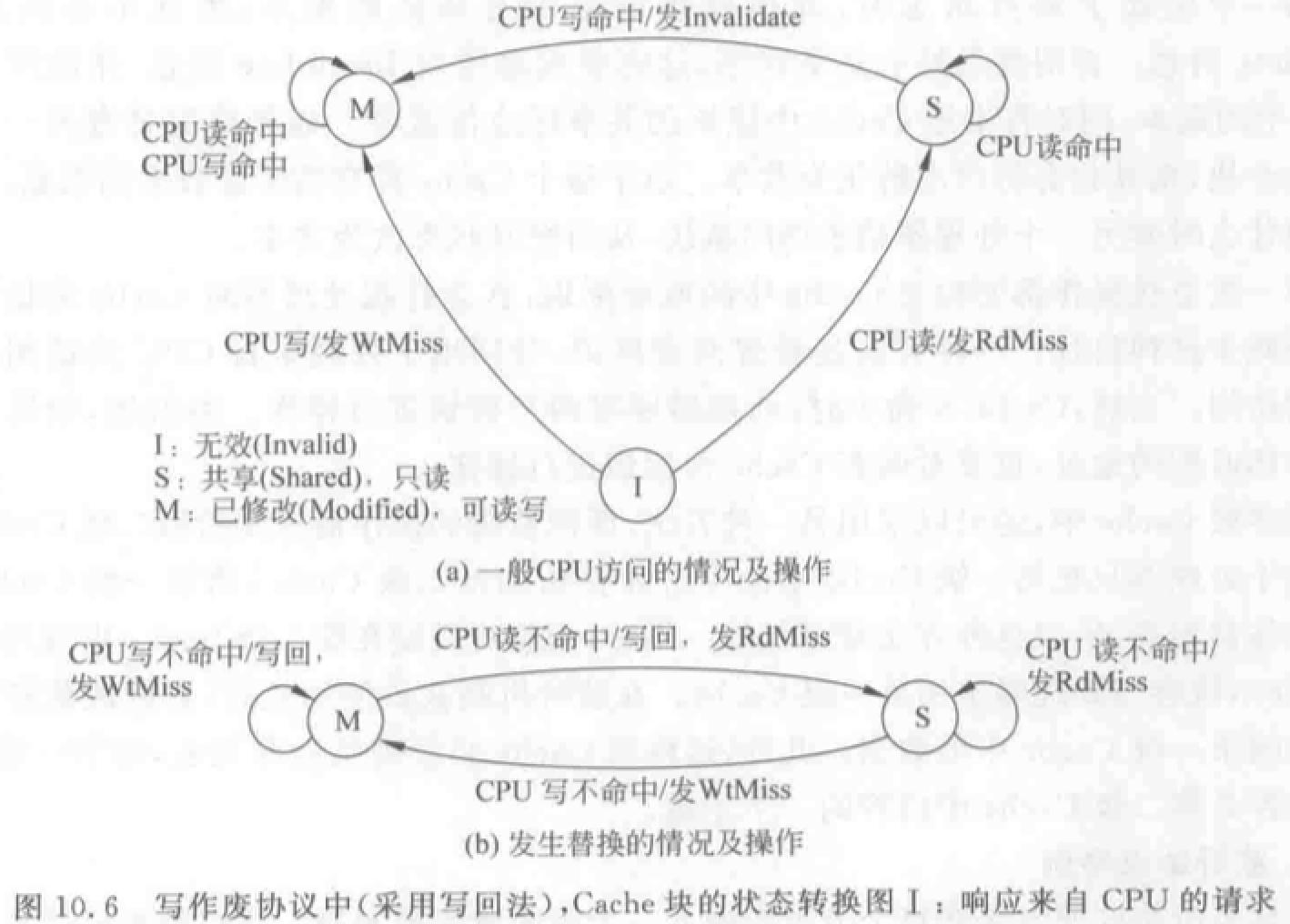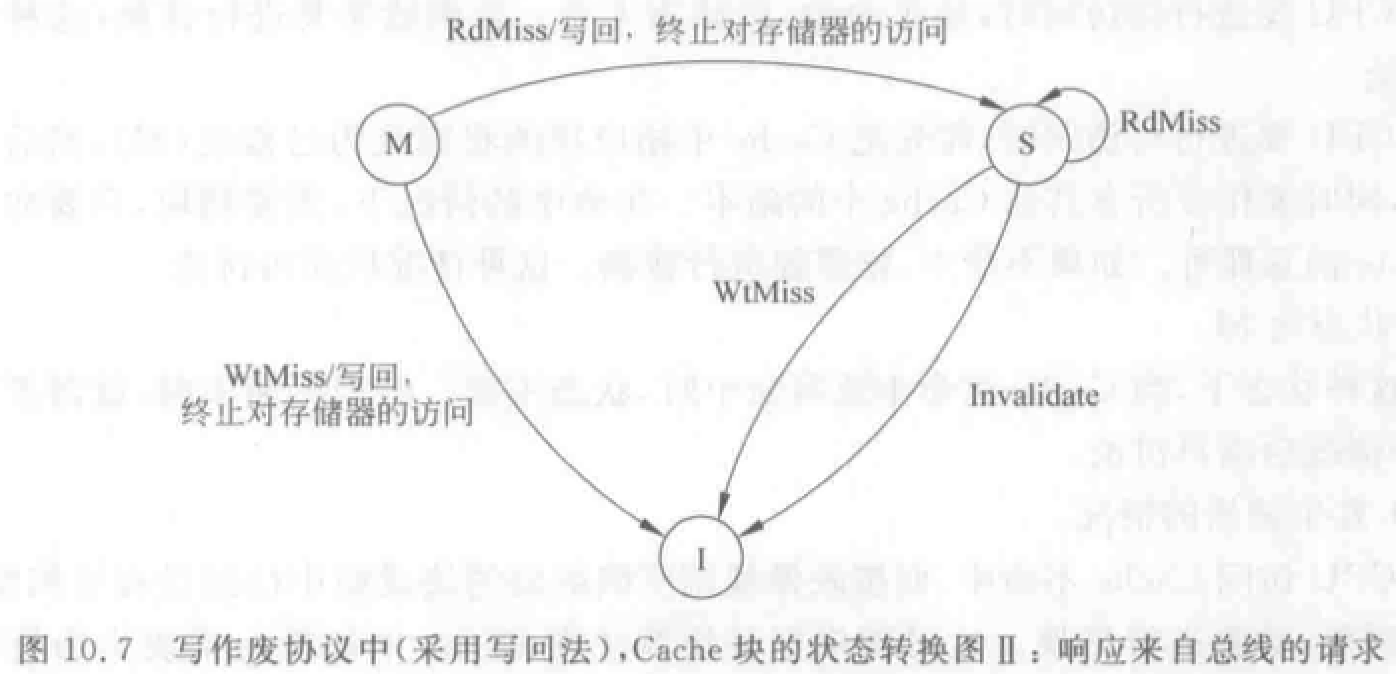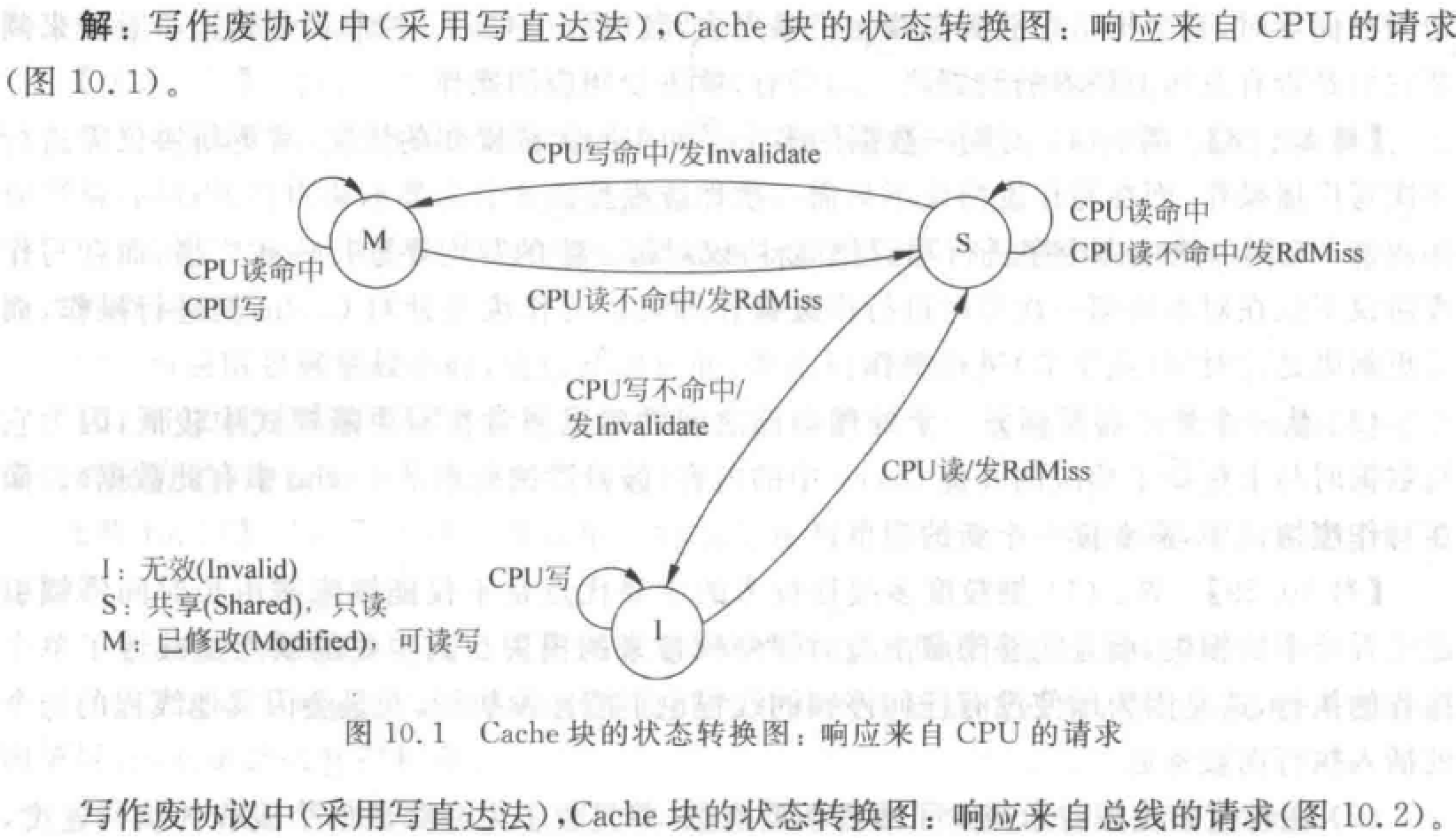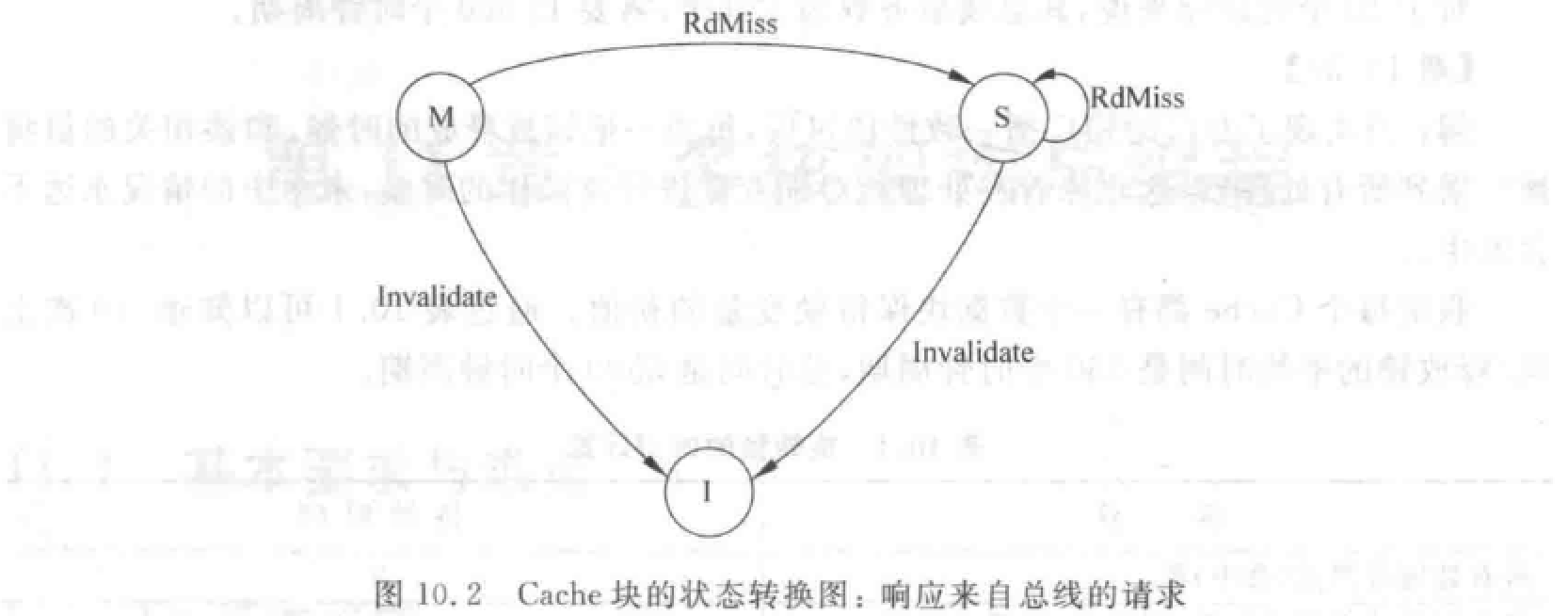## 目录协议的基本思想与实现技术

• 主存中设置一个目录表，存放每个数据块（行）状态位和若干指针位（构成一个位向量），指针位对应处理机 Cache 。
• 状态位指示数据块状态，指针位指出哪些处理机 Cache 中有数据块副本。
• 当一个处理机访问本身 Cache 时，根据目录表，有选择地通知存有数据块的处理机 Cache。避免 Cache 的不一致性。
• 位向量：记录哪些 Cache 中有副本，称为共享集 S。

• 未缓冲：尚未调入 Cache。所有存储器 Cache 中都没有。
• 共享：一个或多个处理机拥有该块副本，且副本与存储器中的该块相同。
• 独占：仅一个处理机有该块副本，且该处理机已经进行了写操作。存储器中该块数据已过时。这个处理机称为该块拥有者。

• 本地结点 A：发出访问请求的结点，假设访问的地址为 K。
• 宿主结点 B：包含所访问的存储单元及其目录项的结点 。
• 远程结点 C：拥有存储块副本的结点。可以和宿主结点是同一个结点，也可以不是。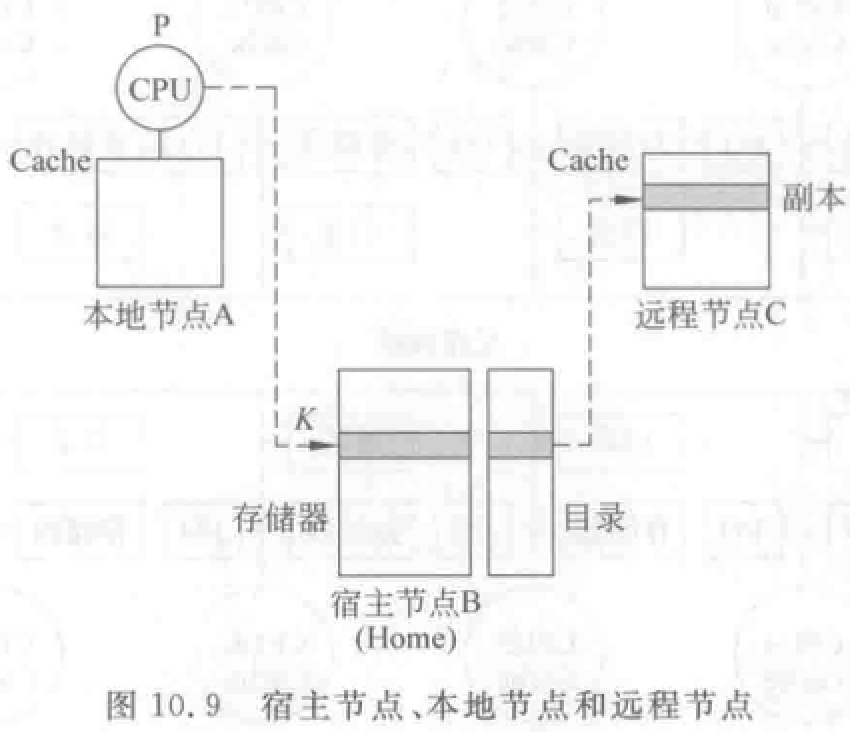• 本地结点发送给宿主结点（目录）的消息：
• RdMiss（P，K）：处理机 P 读地址 k 数据未命中，请求宿主提供该块，并把 P 加入共享集。
• WtMiss（P，k）：处理机 P 写地址 k 数据未命中，请求宿主提供该块，并把 P 设为该块独占者。
• Invalidate（k）：向所有拥有相应数据块副本的远程 Cache 发送消息，作废副本。
• 宿主结点发送给本地结点消息：
• Invalidate（k）：作废远程 Cache 中地址 k 的数据块。
• Fetch（k）：从远程 Cache 取地址 k 数据到宿主结点，并把地址 k 块状态改为共享。
• Fetch&Invalidate（k）：从远程 Cache 取地址 k 数据到宿主结点，并作废远程 Cache 中该块。
• 远程结点发给宿主结点的消息：
• WtBack（k，D）：把远程 Cache 中地址 k 数据块 D 写回到宿主结点。这是对 Fetch 或 Fetch&Invalidate 的响应。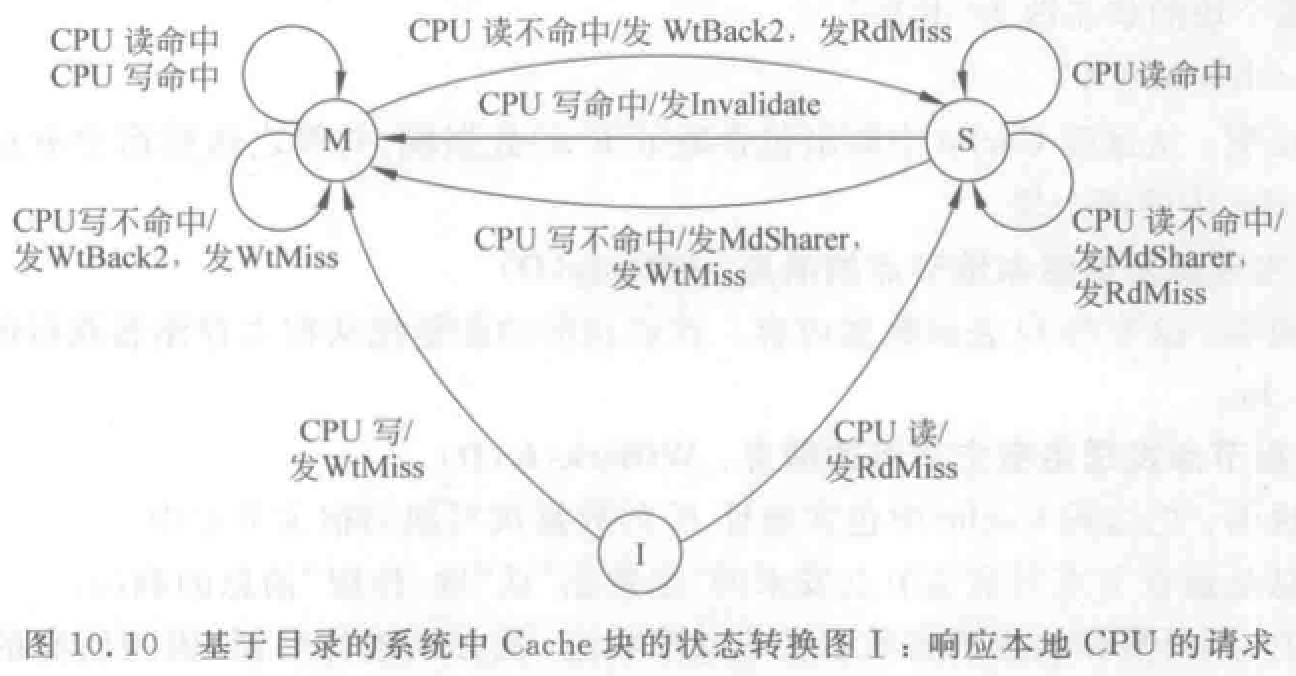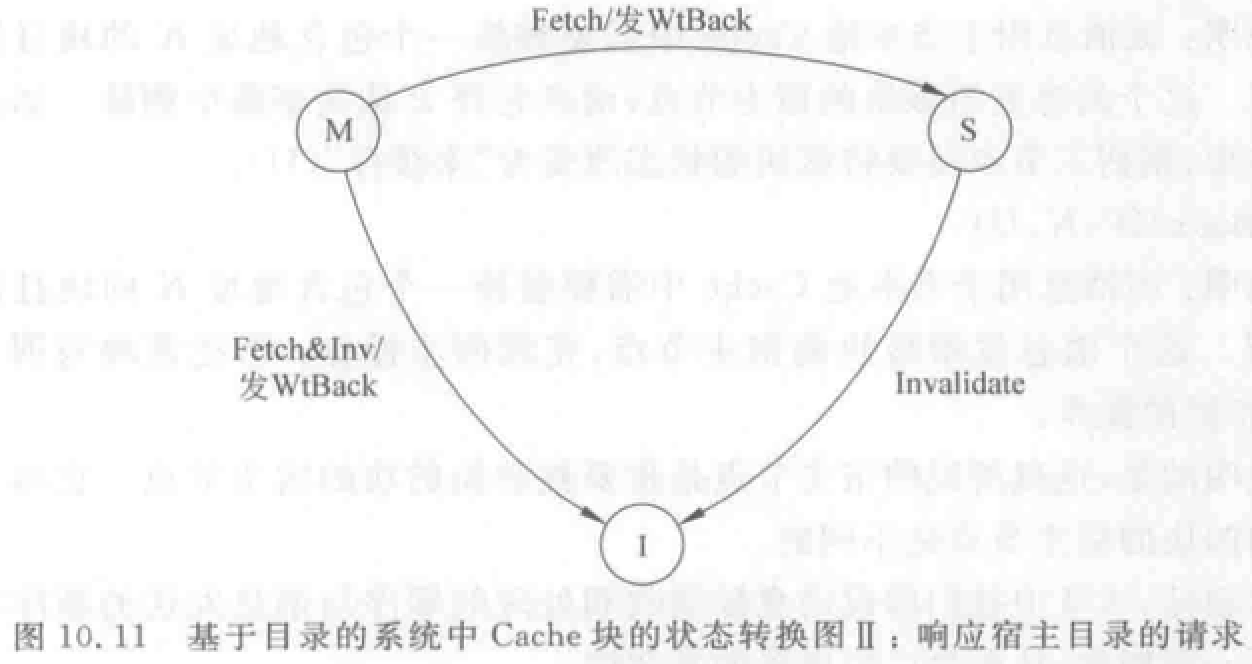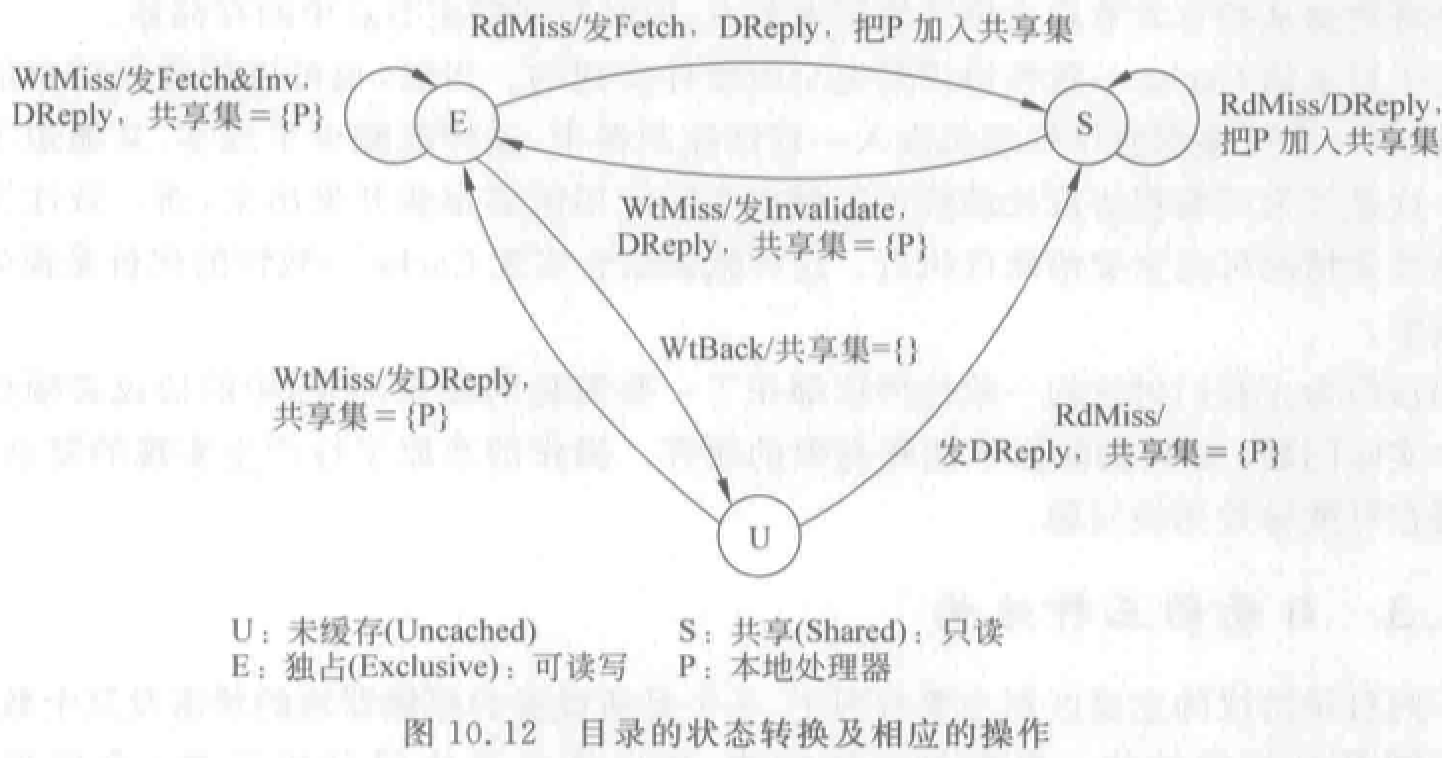• 全映像目录：最大。

• 每个目录项包含 $N$ 位位向量，对应处理机个数。
• 优点：① 处理比较简单、速度快。② 每个 Cache 允许存放同一数据块的副本。
• 缺点：① 存储空间开销大。② 目录空间复杂度$O(N^2)$。③ 可扩放性很差。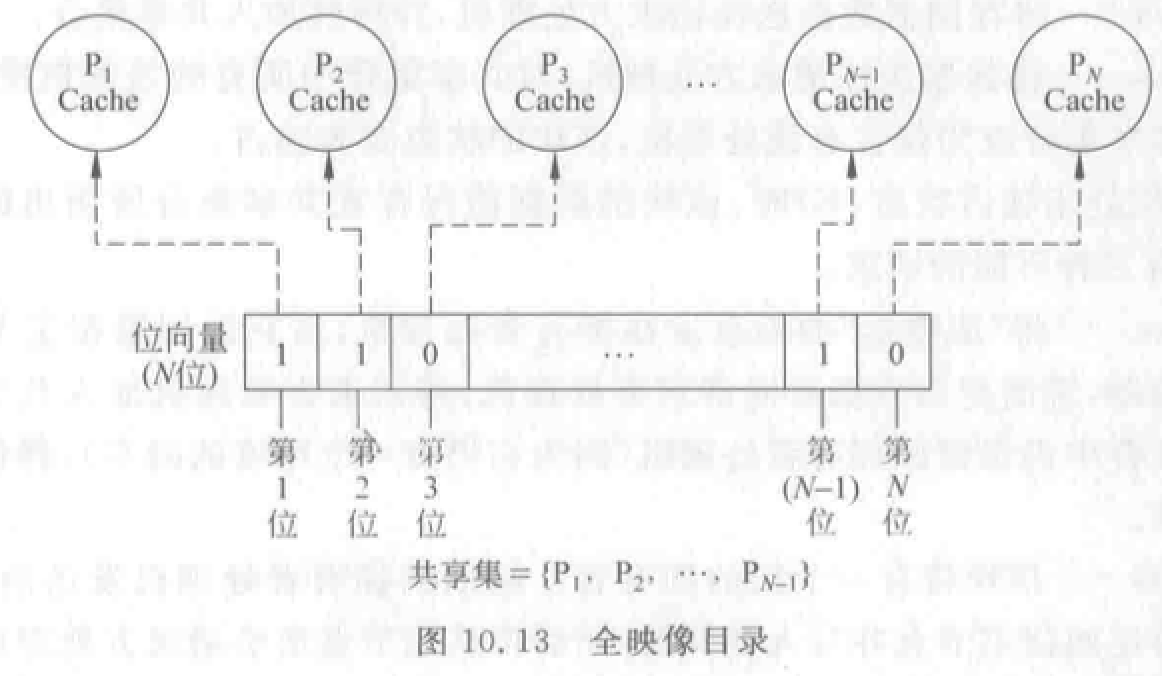• 有限映像目录：较大。

• 优点：提高可扩放性，减少目录占用空间。

• 核心思想：采用位数固定的目录项目。限制同一数据块在所有 Cache 中的副本总数。

• 缺点：当目录项中 m 个指针占满，而又需要新调入该块时，就需要在 m 个指针中选择一个废弃。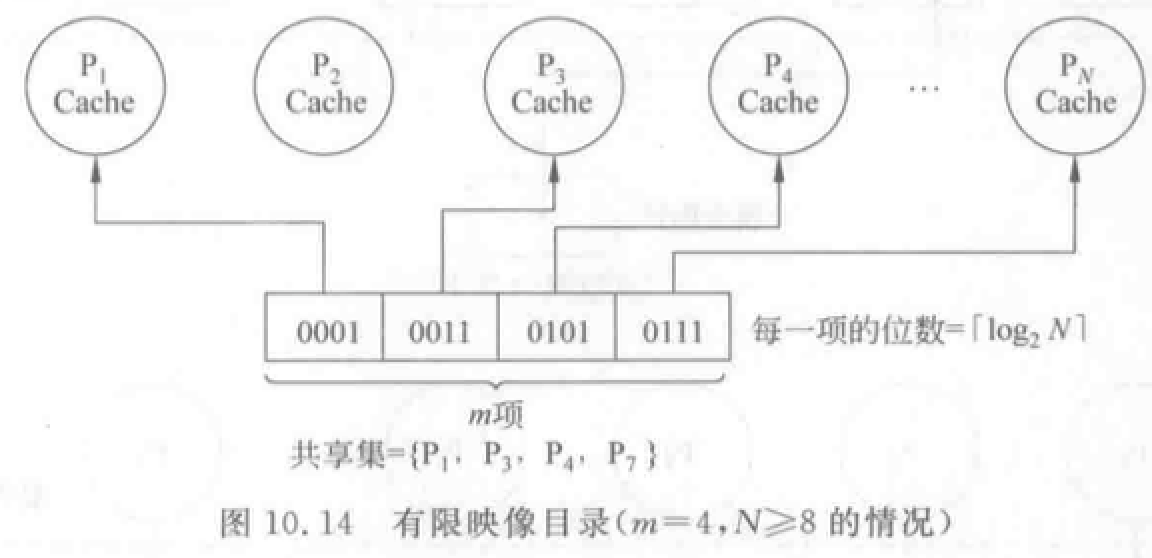• 链式目录：最小。

• 用一个目录指针链表表示共享集合。主存只有一个指针位指向一个处理机 Cache。

• 优点：不限制副本个数，扩展性好。# 第十三章 阵列处理机

• 分布式存储器的阵列机
• 共享存储器的阵列机

• 递归折叠求和算法

## 概念

• SIMD
• 利用并行性中的同时性，而非并发性。所有处理单元同时进行相同的操作。
• 受互连网络结构限制，限制了适用的求解算法类别。

## 结构

• 分布式存储器阵列机：

• 主机：程序编辑、编译、调试，程序和数据装入（控制存储器）。
• 指令送到阵列控制部件译码。标量指令由标量处理机执行，向量指令通过广播总线播送到所有 PE 执行。
• 数据集划分后通过数据总线分布存放到 PE 的本地存储器 LM。
• 有多个处理单元 PE，PE 有自己的本地存储器 LM。PE 之间通过数据寻径网络连接实现通信。
• PE 的同步在控制部件的控制下由硬件实现，PE 在一个周期执行同一条指令。
• 屏蔽逻辑控制某些 PE 在指定指令周期是否参与执行。
• 主要区别在于数据寻径网络不同。IlliacIV：4 邻接网络、CM-2：嵌入网格的超立方体，等。
• 大部分阵列处理机都是分布式存储器。易于构成 MPP。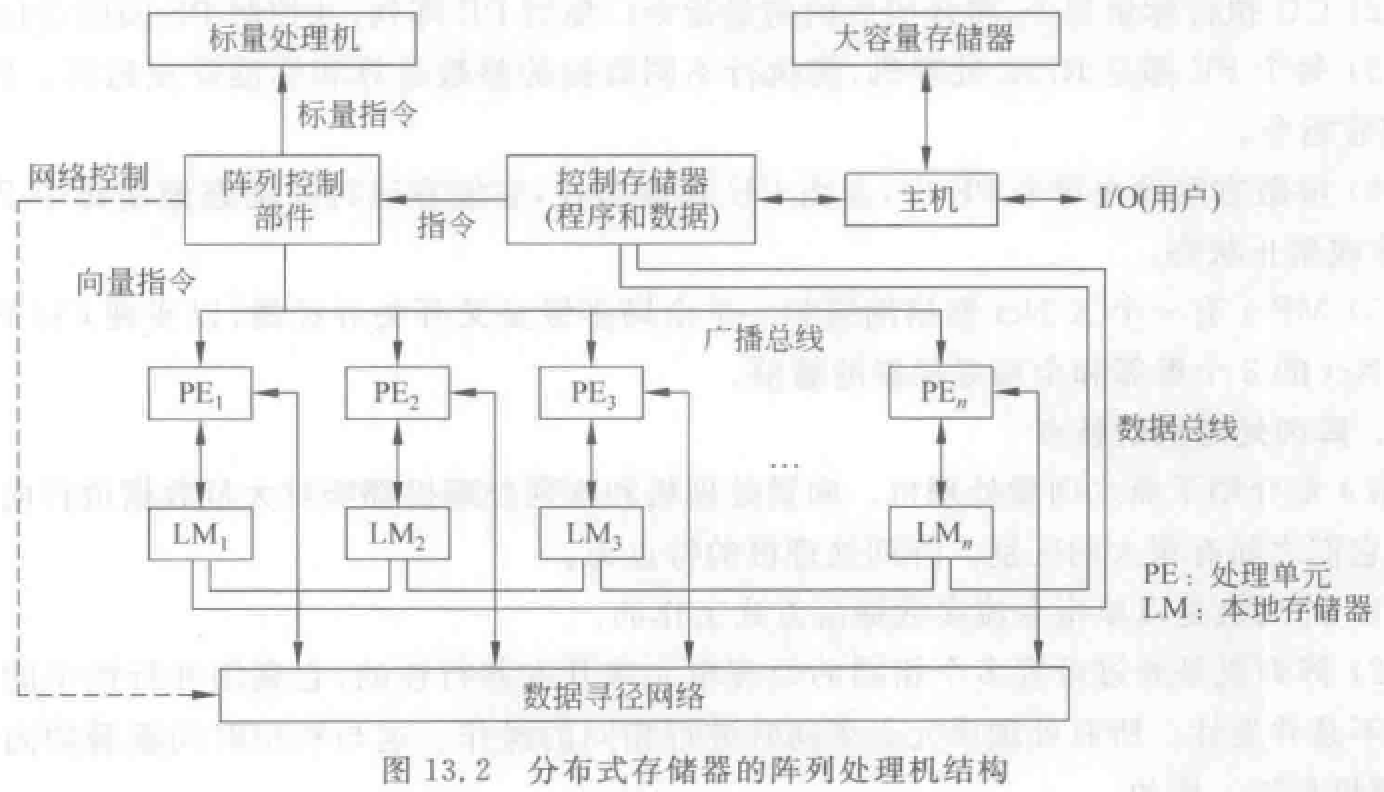• 共享存储器

• 集中设置存储器：共享多体并行存储器 SM——通过对准网络——连接 PE。
• 存储模块数目等于或略大于 PE 数目。
• 必须减少访存冲突，适合较少 PE 情况。
• 所有阵列指令使用长度等于 PE 个数的向量操作数。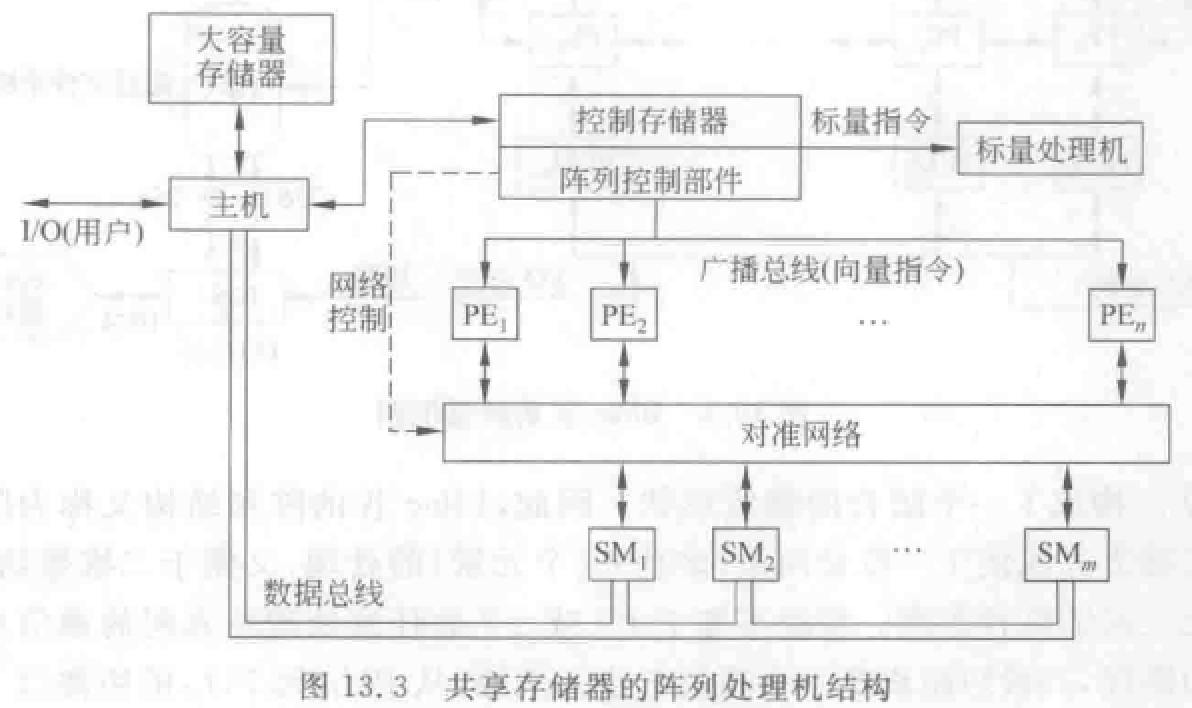## 类 BSP 机存储体分配

• 先将二维数组按列优先或者按行优先的顺序变换为一维数组，以形成一个一维线性地址空间，地址用 $A$ 表示。
• 然后将地址 $A$ 变换成并行存储器地址 $(i,j)$。其中$j$ 是存储体体号，$j=A \pmod M$$i$ 是在相应存储体内的地址，$i=\lfloor \frac AN \rfloor$。存储体的个数 $M$ 是一个质数。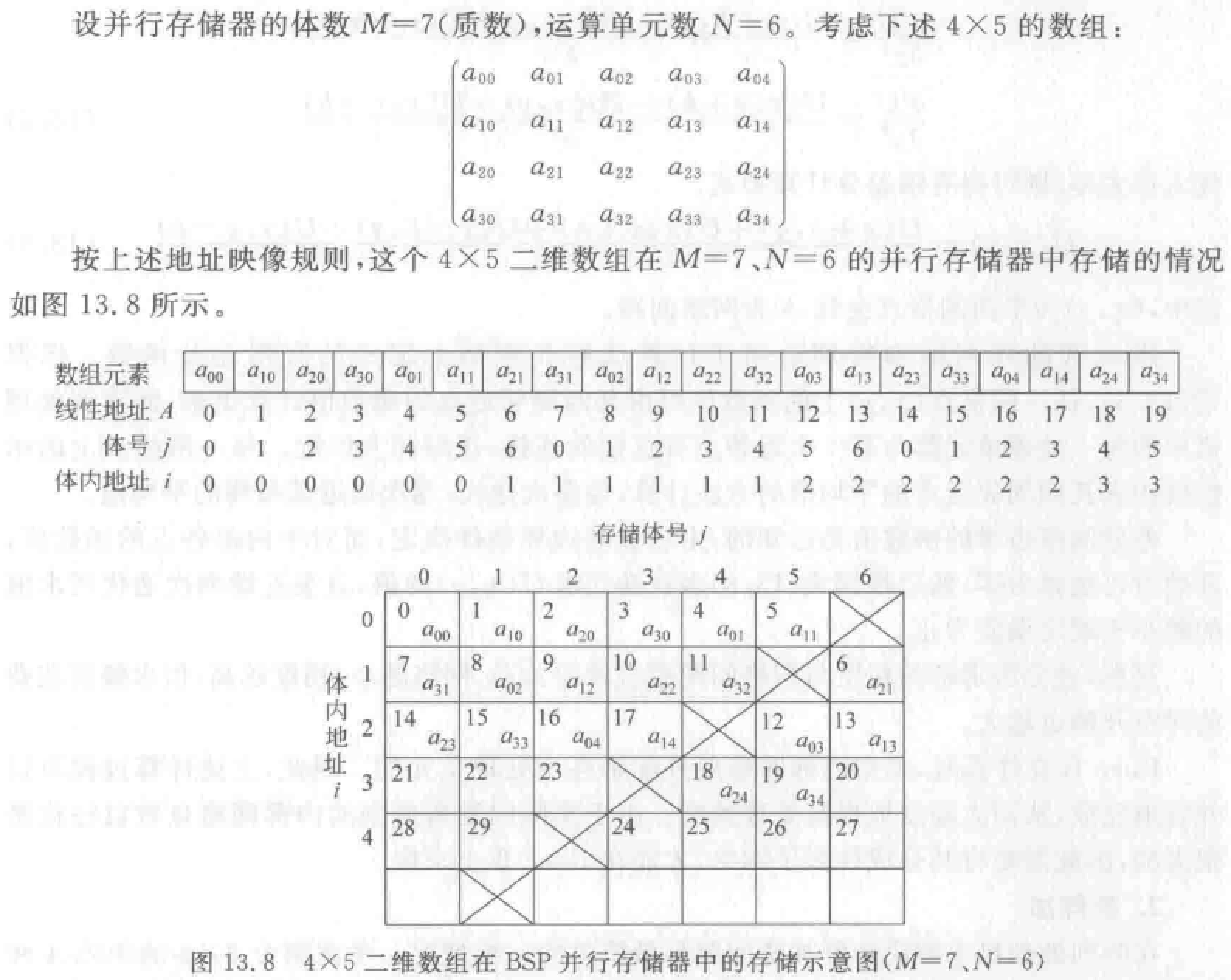## 阵列处理机与流水线向量处理机对比

• 均适合高速数值计算。阵列处理机有固定结构，与一定算法相联系。
• 阵列处理机用大量处理单元对各分量运算，各单元同时进行相同操作，依赖空间并行技术。并行化程度高，潜力大。
• 向量处理机基于流水线技术，时间并行性
• 阵列处理机每个单元负担多种处理功能，相当于向量机多功能流水部件，处理数据效率较低。
• 互连网络设计和研究——阵列机研发重点。
• 阵列机有一台标量处理机和一台前端机，向量处理部件是系统主体。机器性能主要取决于向量处理部分。也取决于标量运算速度和编译开销。

## 算法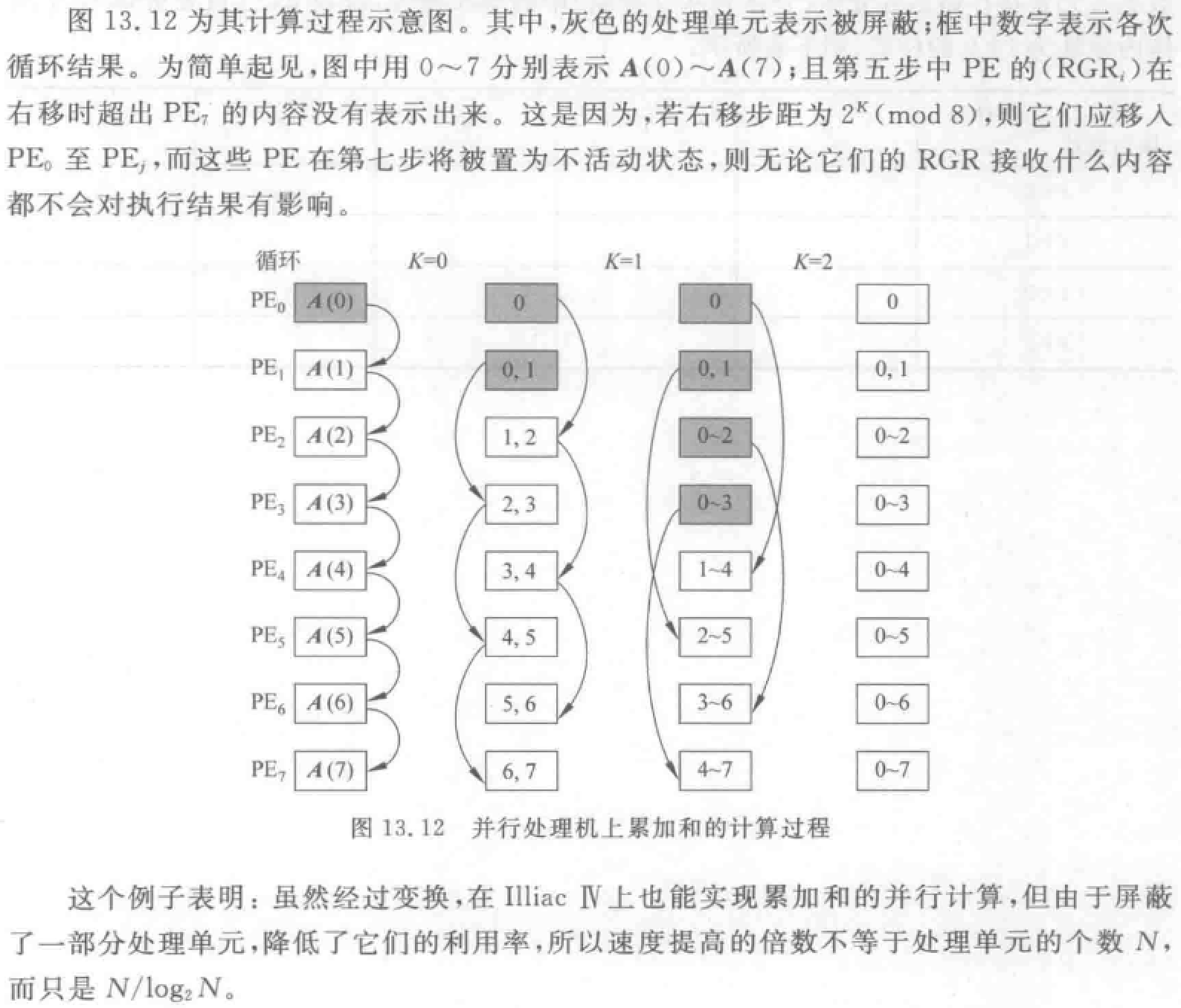• 标量 a 广播时间 = $传送时间 2\Delta t \times 网络直径 3 = 6\Delta t$
• 阵列处理器处理时间 两次加法一次乘法 = $2 \times 1\Delta t + 4\Delta t = 6\Delta t$
• 递归折叠时间 传+乘 传+乘 传+乘 = $2+4+2+4+2+4 = 18\Delta t$

1. 在 SISD 机上计算 S 时间？

2. 在超立方体结构上计算 S 的时间是多少？

（1）在 SISD 机上计算 s 需要串行计算 32 次乘法和 31 次加法。共需要时间：$32\times 4\Delta t+31\times 2\Delta t=190\Delta t$

（2）共有 16 个 PE，每个 PE 各自有 $A_i$$B_i$ 对两组。

https://blog.xqmmcqs.com/计算机系统结构复习笔记 - 第九、十、十三章/

xqmmcqs

2022-06-09

2022-07-11